# RD Sharma Solutions for Class 12 Maths Chapter 2 Functions

## RD Sharma Solutions for Class 12 Maths Chapter 2 – Free PDF Download Updated for (2021-22)

RD Sharma Solutions Class 12 Maths Chapter 2 Functions help students who aspire to obtain a good academic score in the exam. The solutions are designed by experts to boost confidence among students in understanding the concepts covered in this chapter and methods to solve problems in a shorter period. Students can access RD Sharma Solutions while practising problems for step-by-step guidance and instant doubt clearance.

Chapter 2 Functions of Class 12 RD Sharma Solutions explains the function, domain and codomain of functions. It has four exercises. RD Sharma Solutions for Class 12 resources are prepared based on the Class 12 CBSE syllabus by our subject experts, taking into consideration, the types of questions asked in CBSE Class 12 board exam. Students can easily access answers to the problems present in RD Sharma Solutions for Class 12. Let us have a look at some of the important concepts that are discussed in this chapter.

• Classification of functions
• Types of functions
• Constant function
• Identity function
• Modulus function
• Integer function
• Exponential function
• Logarithmic function
• Reciprocal function
• Square root function
• Operations on real functions
• Kinds of functions
• One-one function
• On-to function
• Many one function
• In to function
• Bijection
• Composition of functions
• Properties of the composition of functions
• Composition of real function
• Inverse of a function
• Inverse of an element
• Relation between graphs of a function and its inverse

## Download the PDF of RD Sharma Solutions For Class 12 Maths Chapter 2 Functions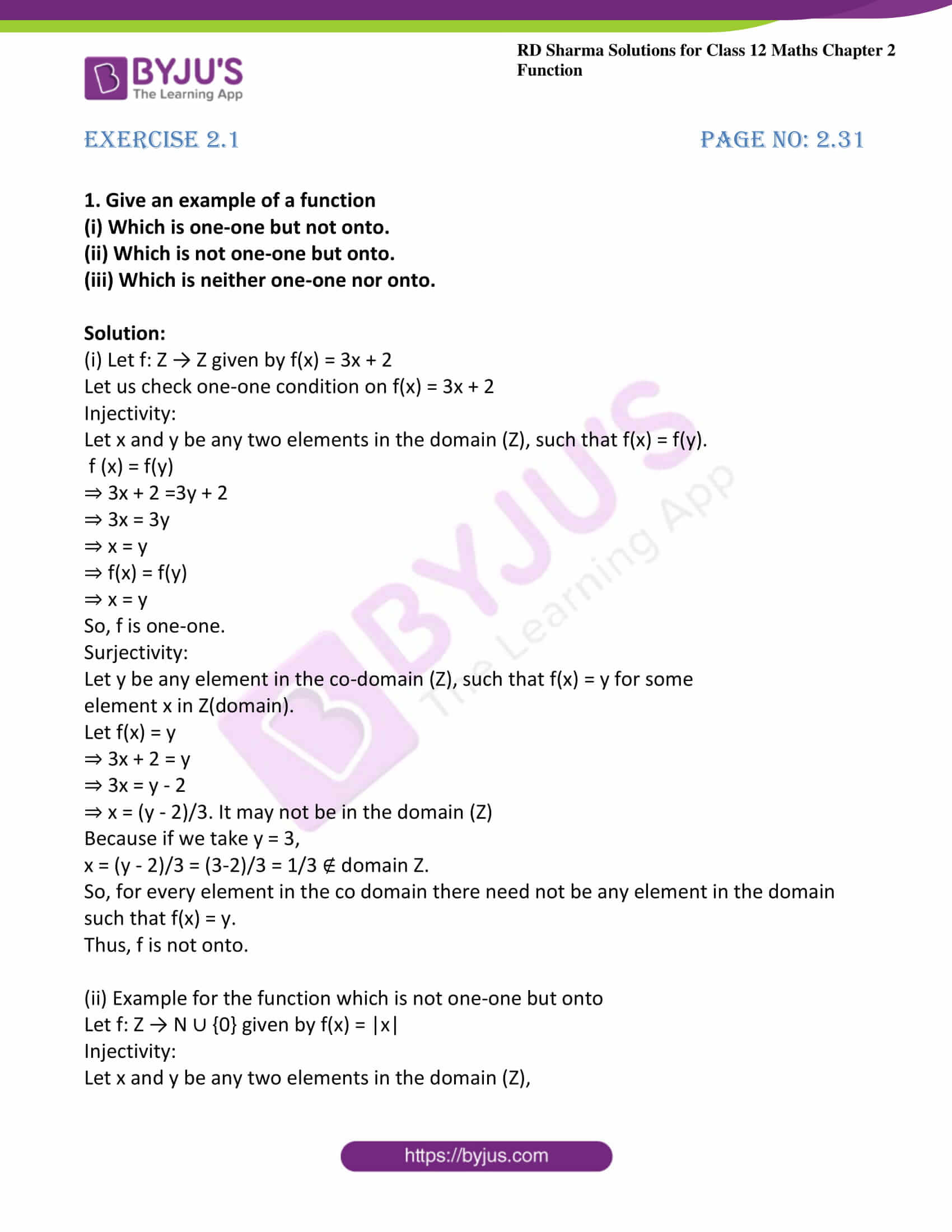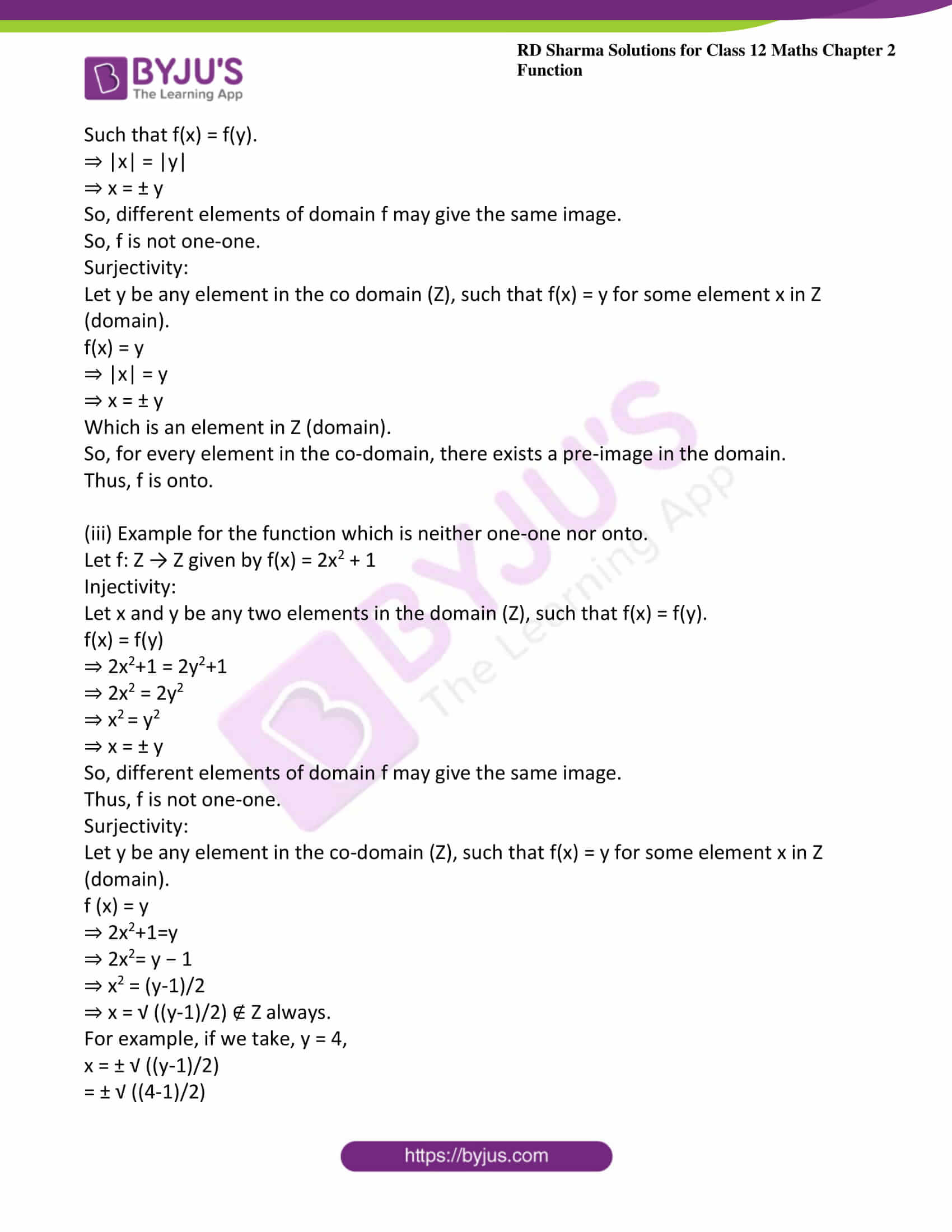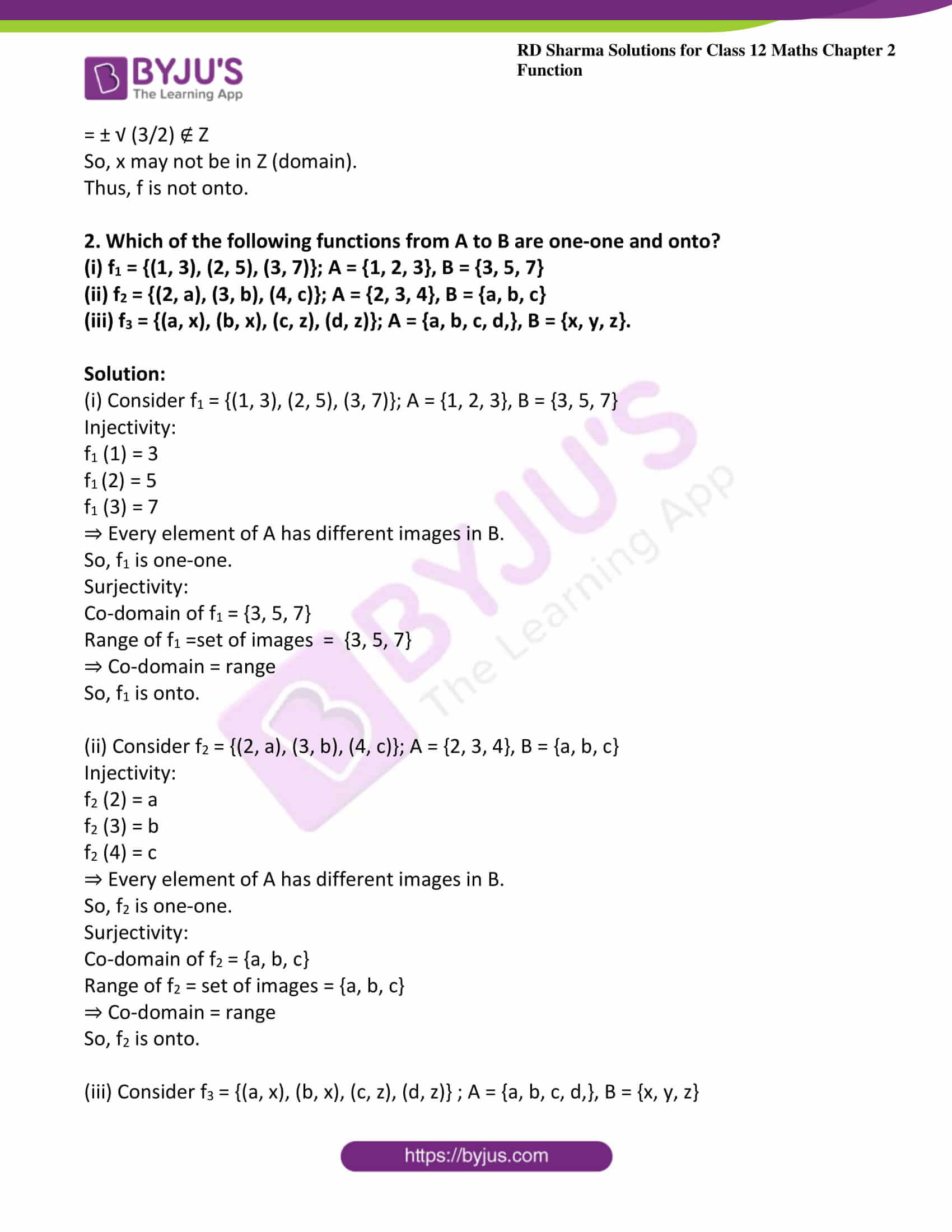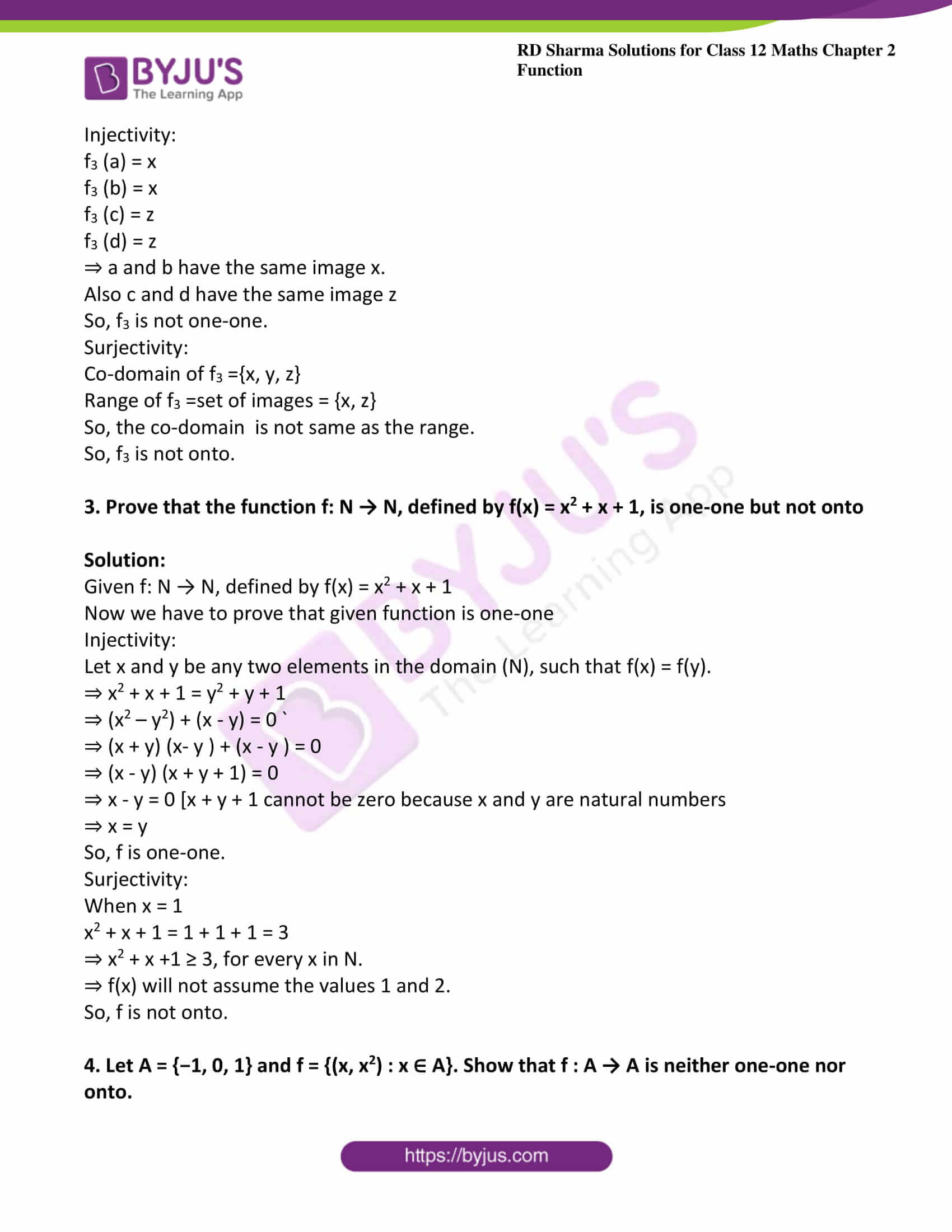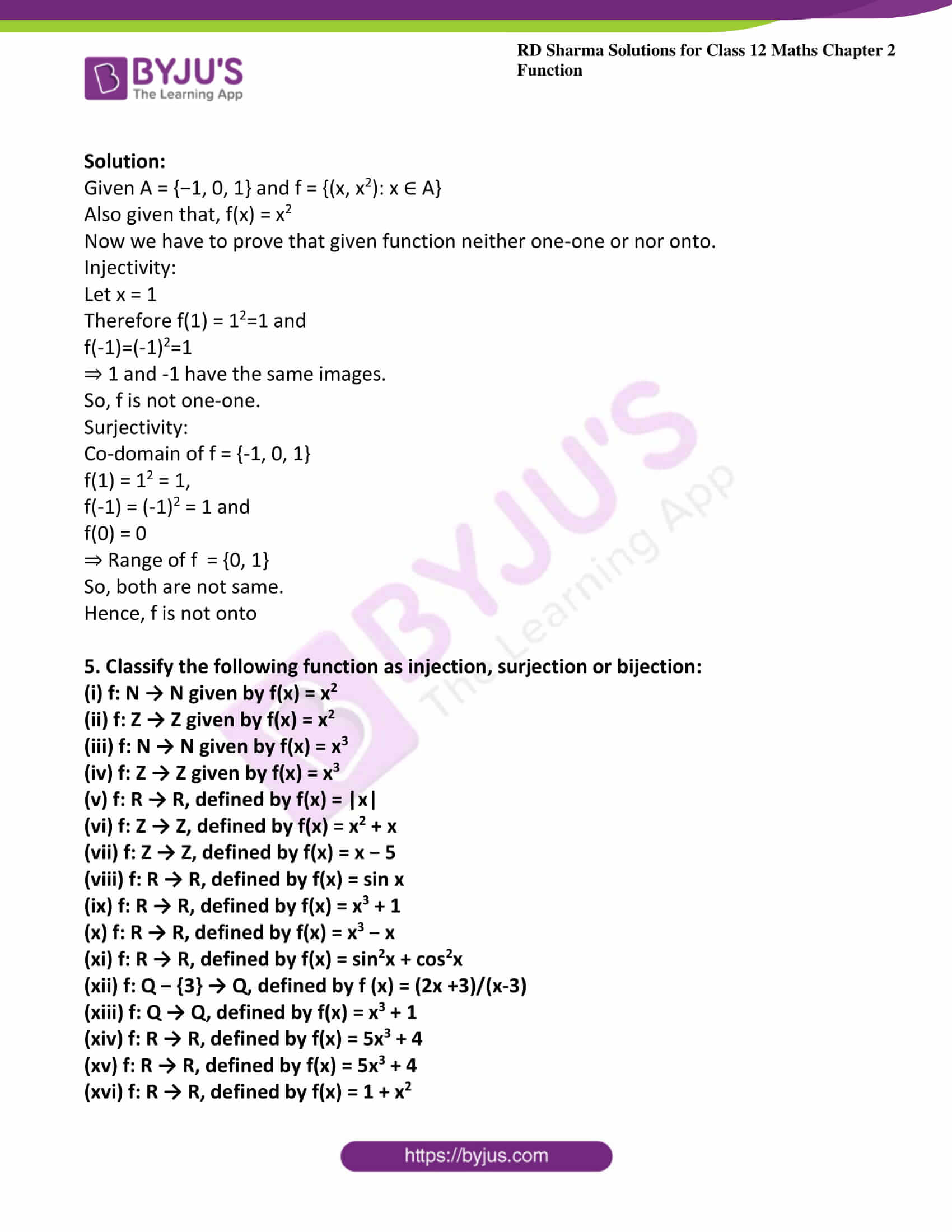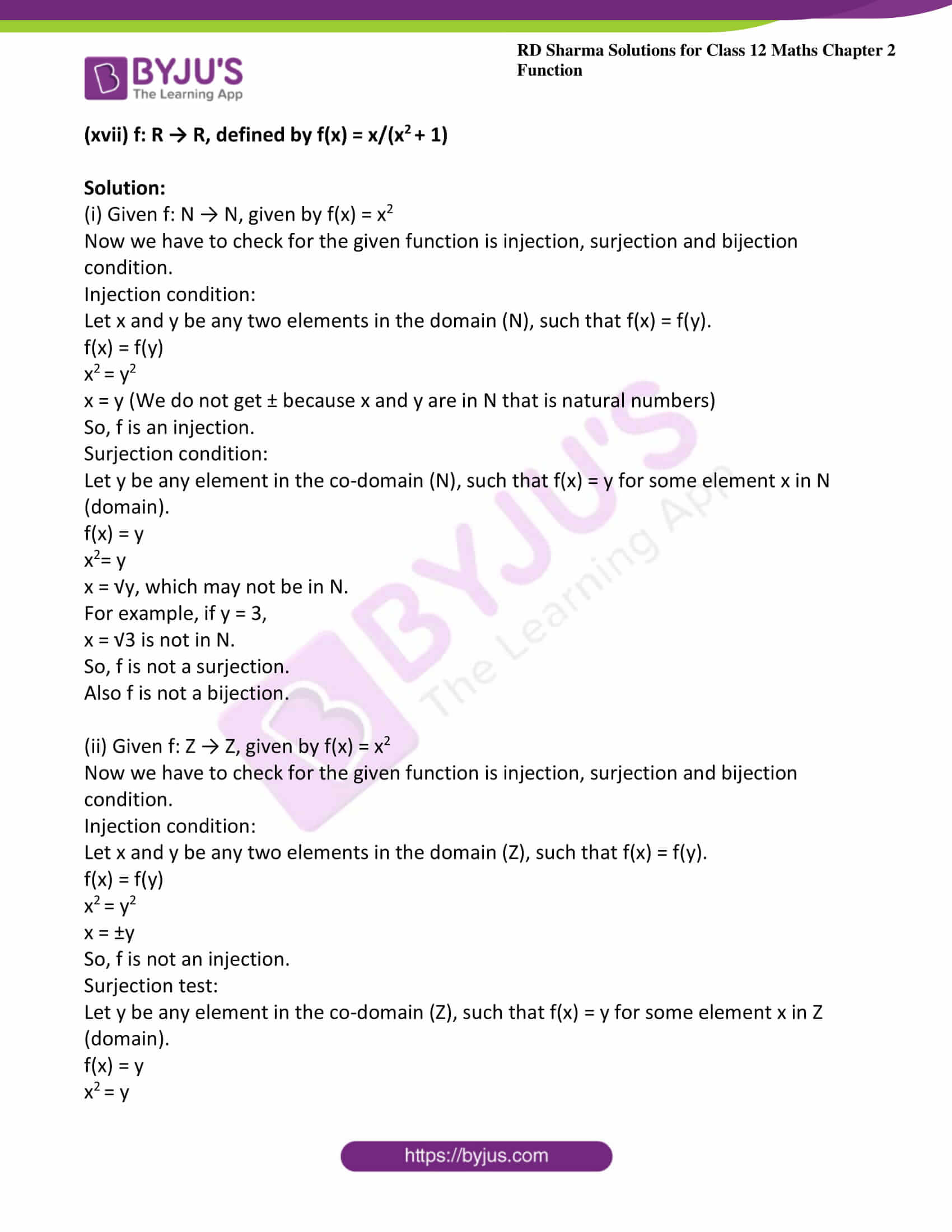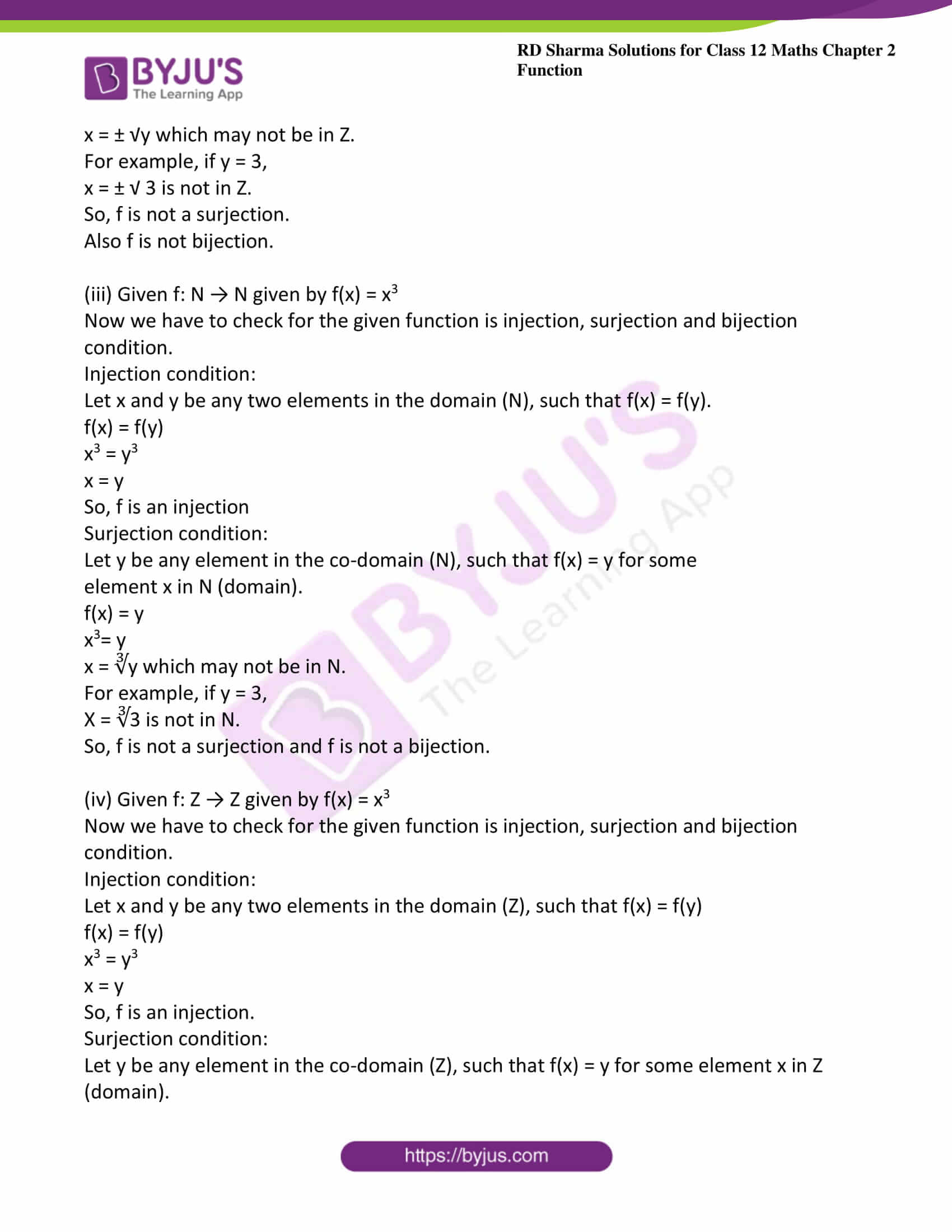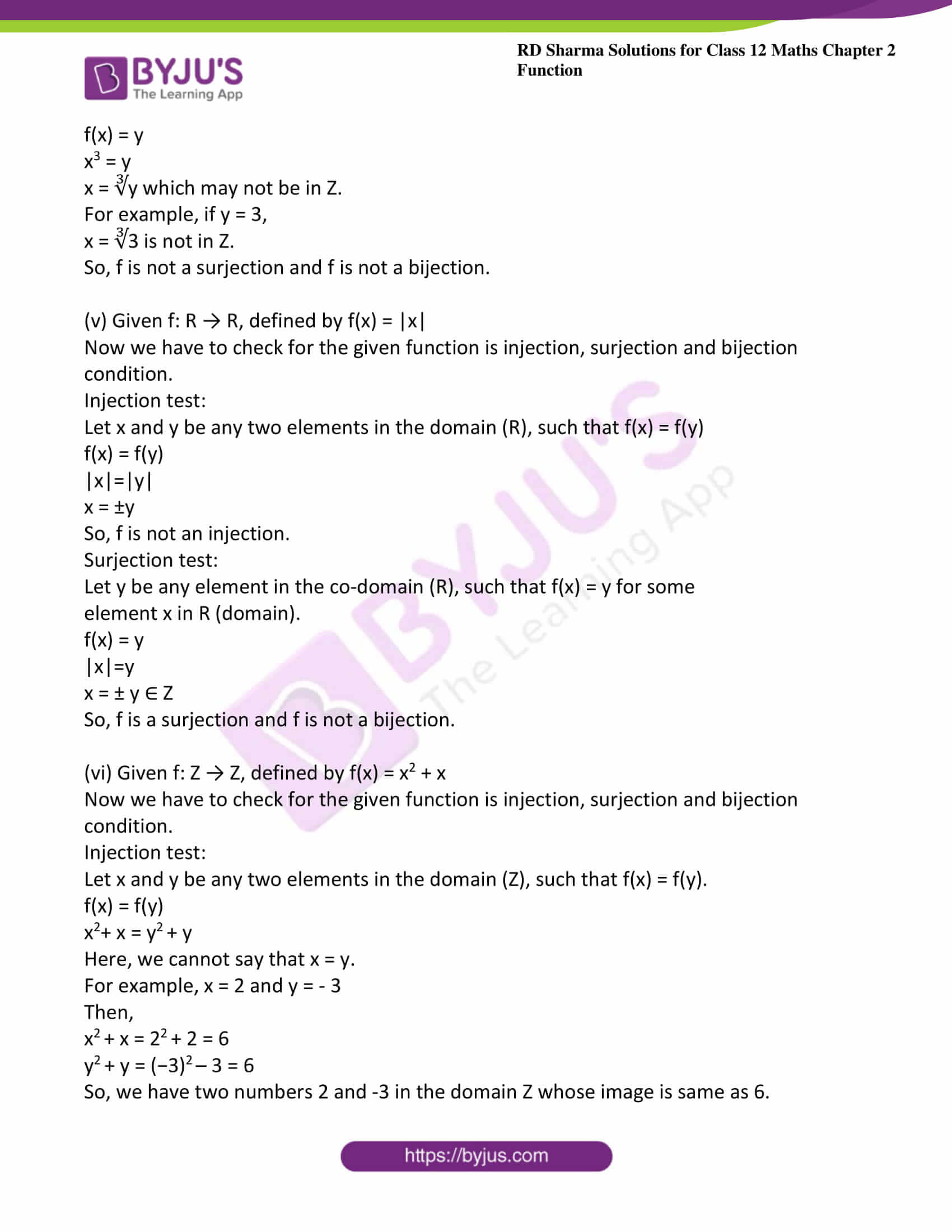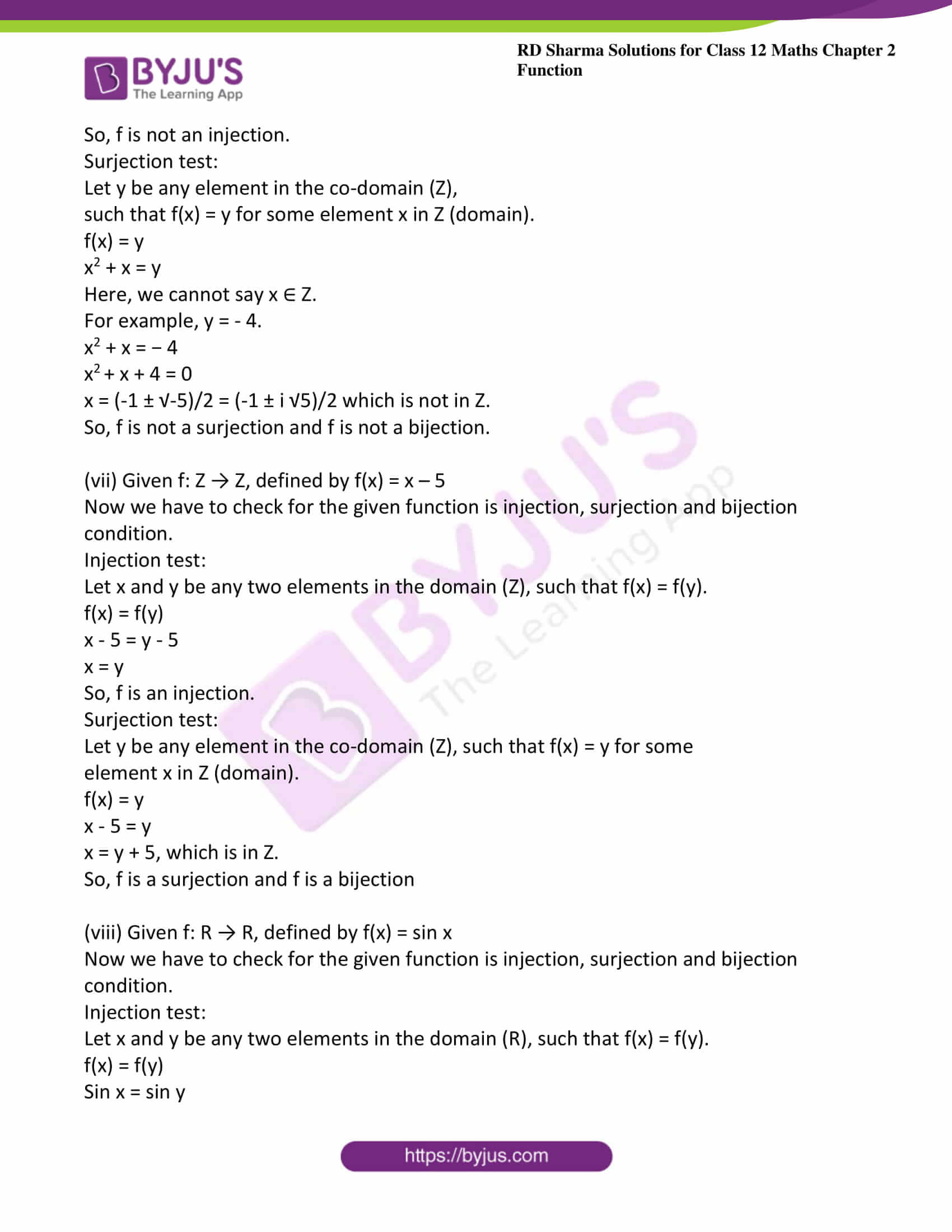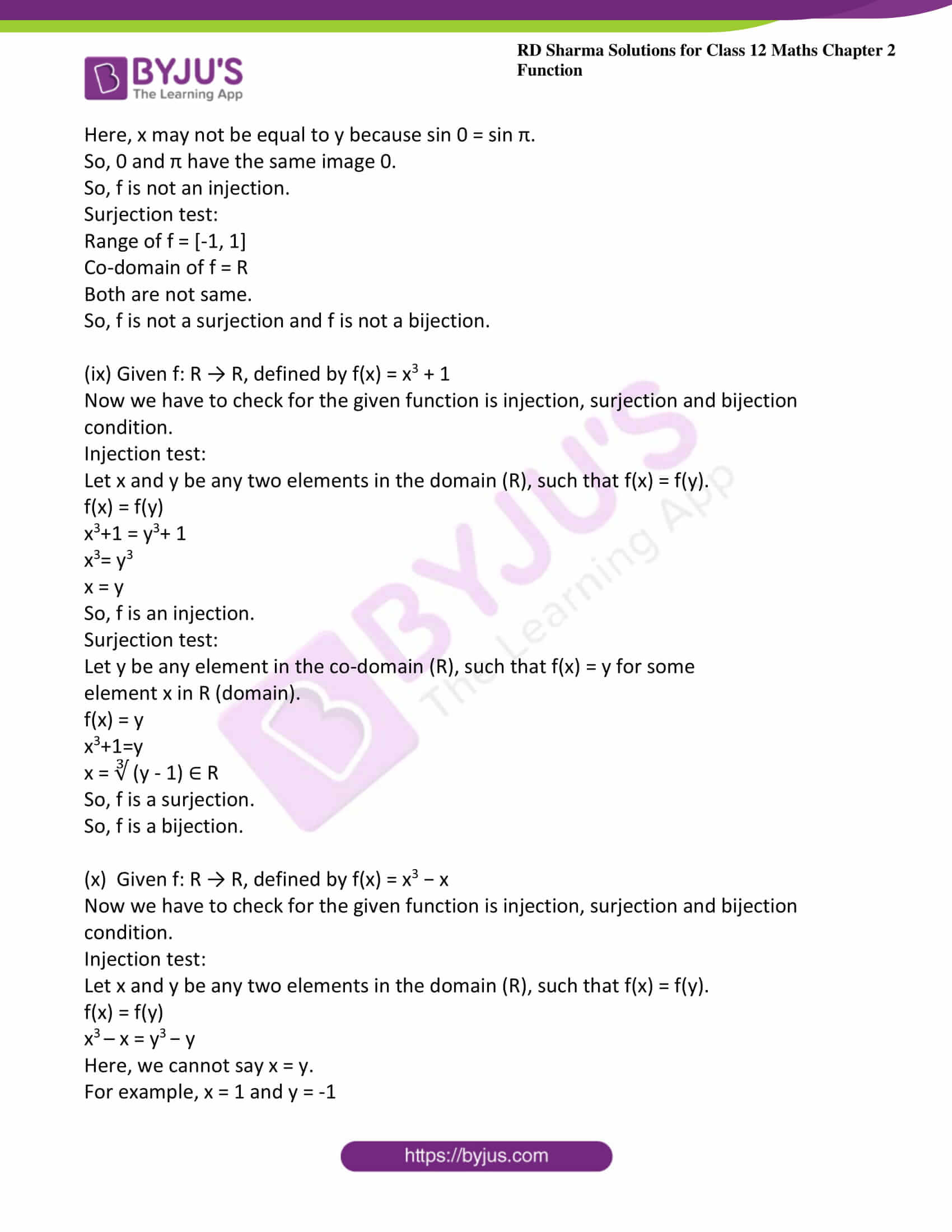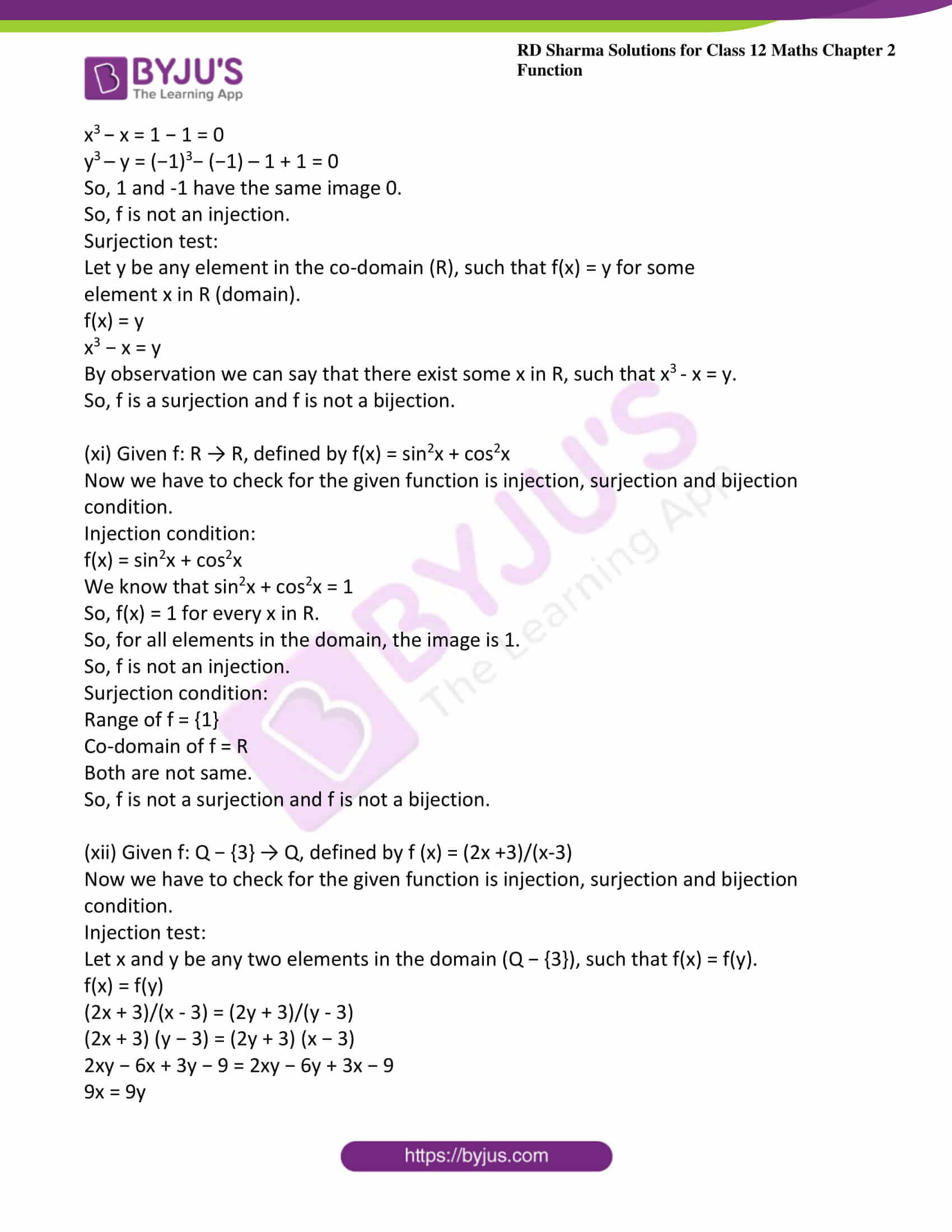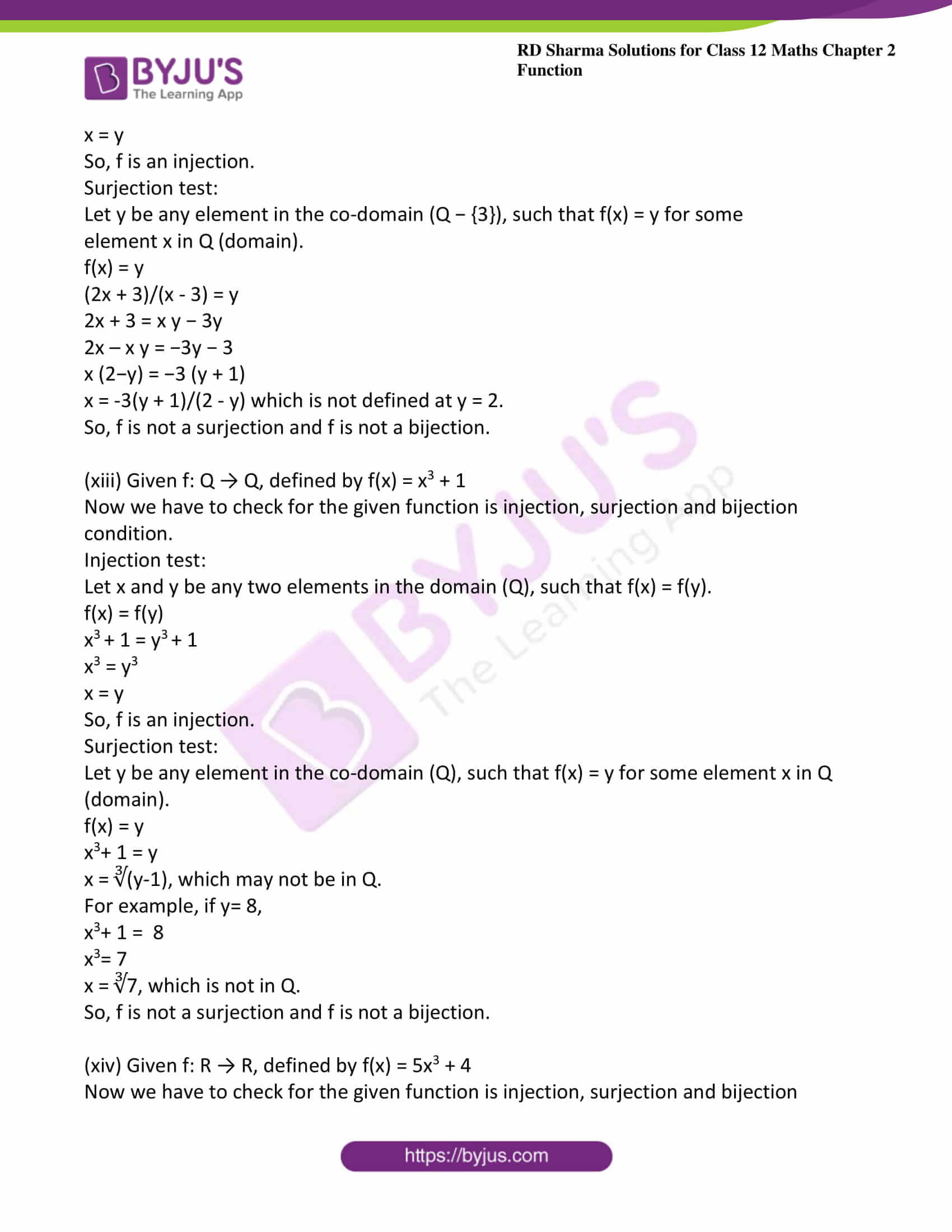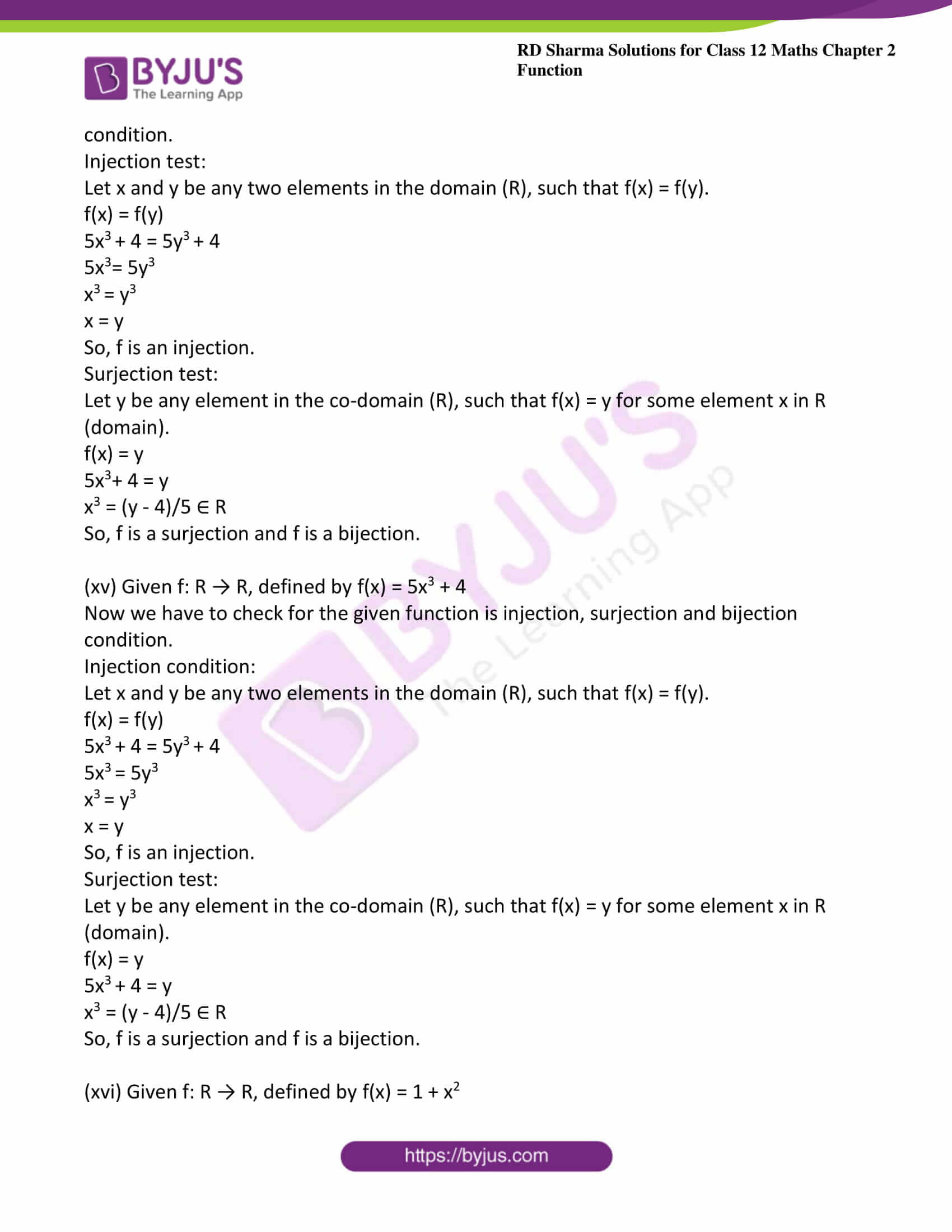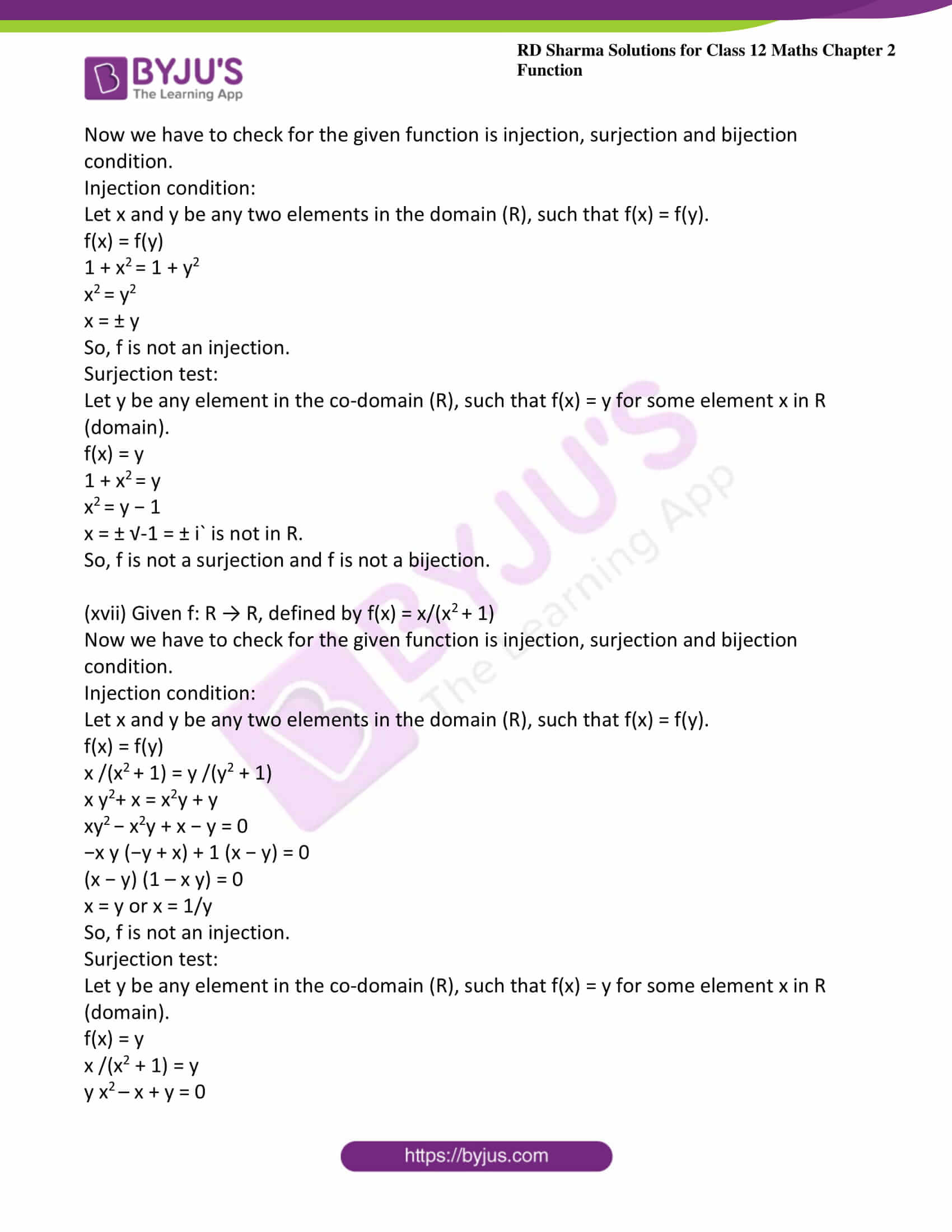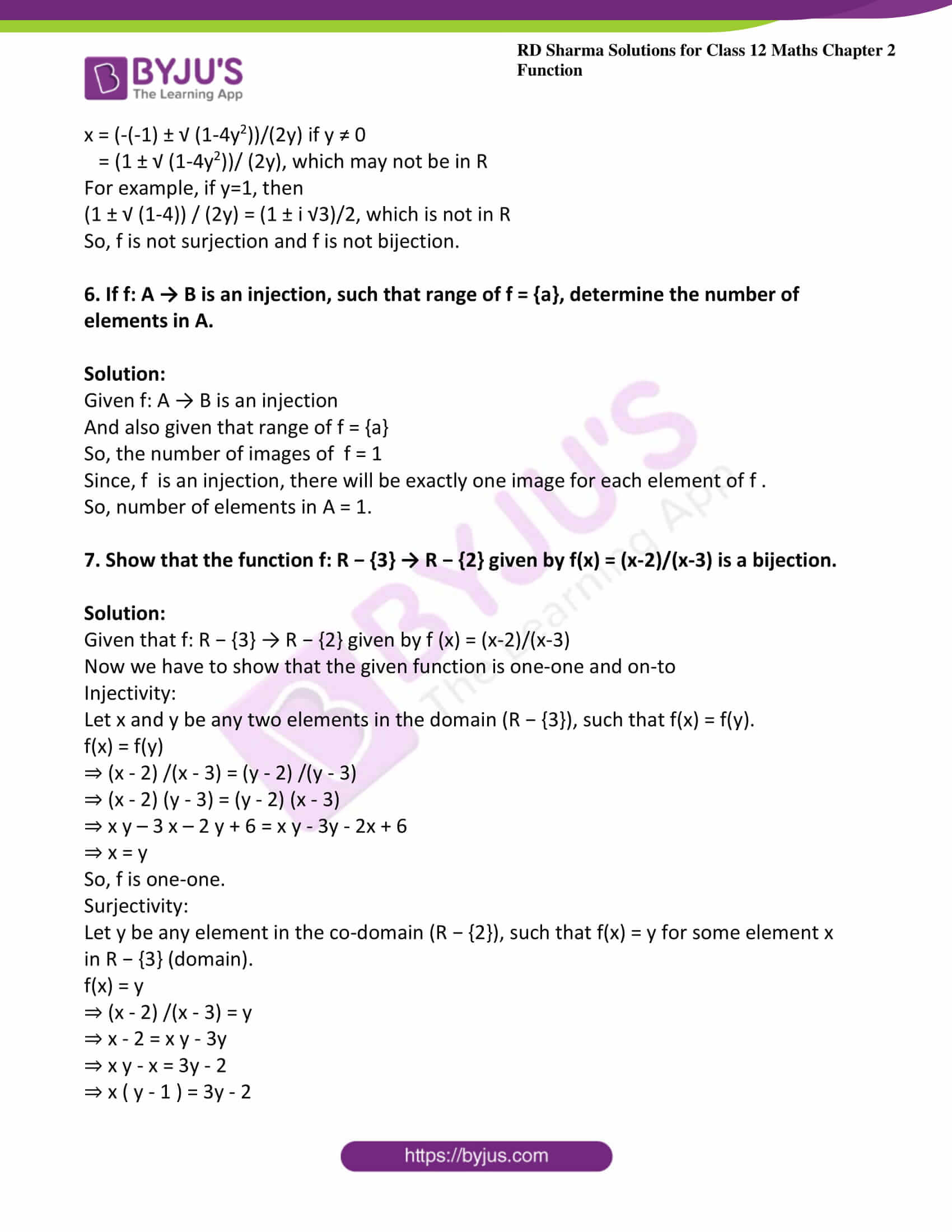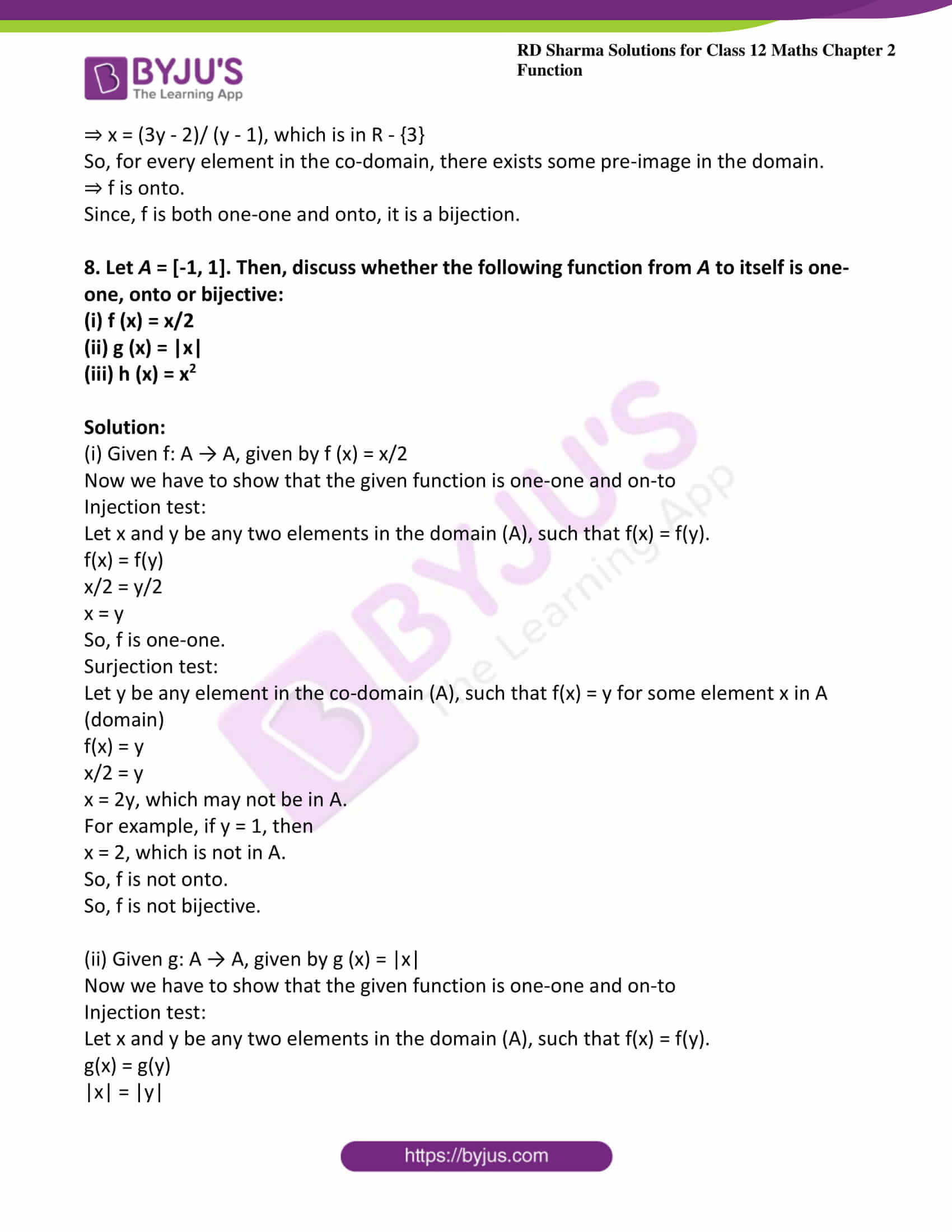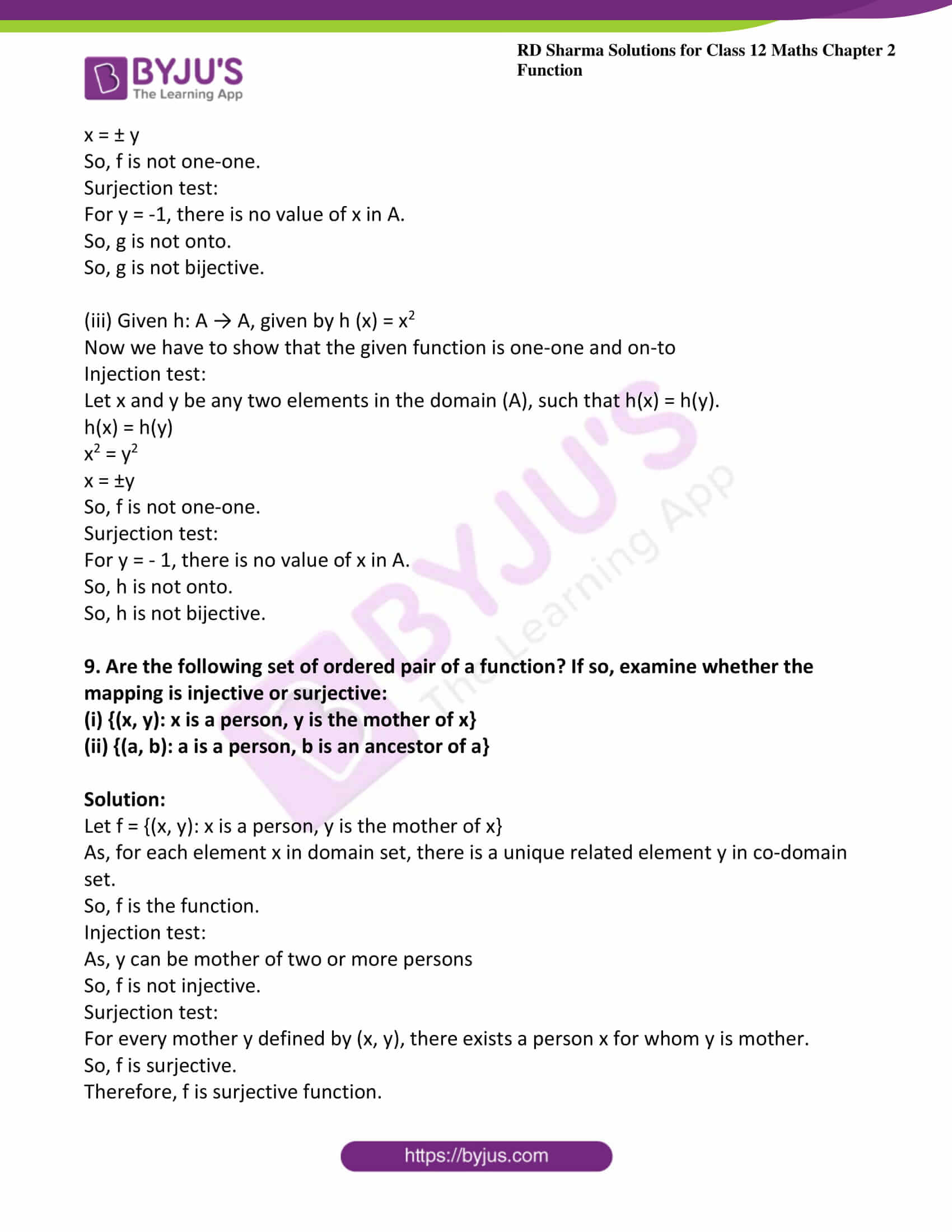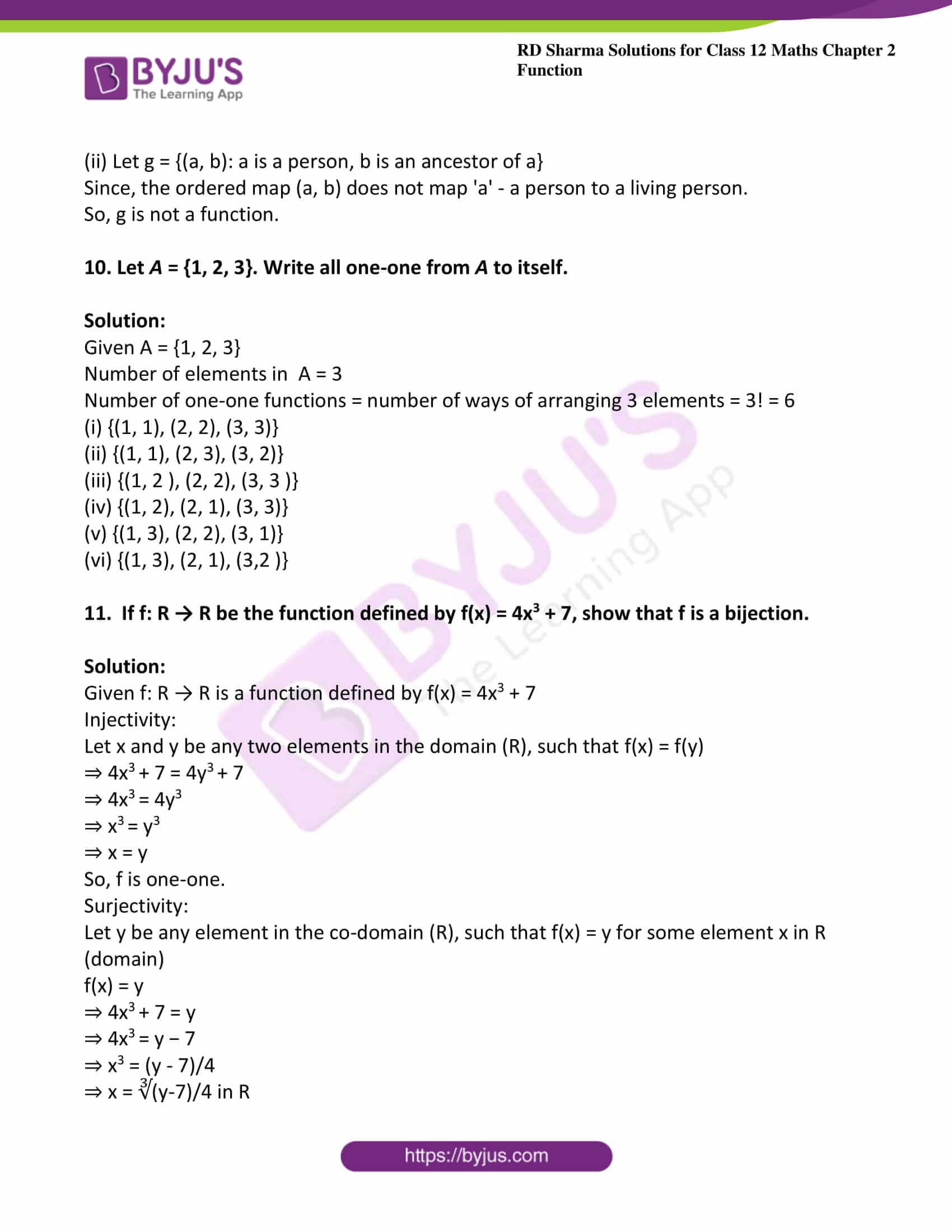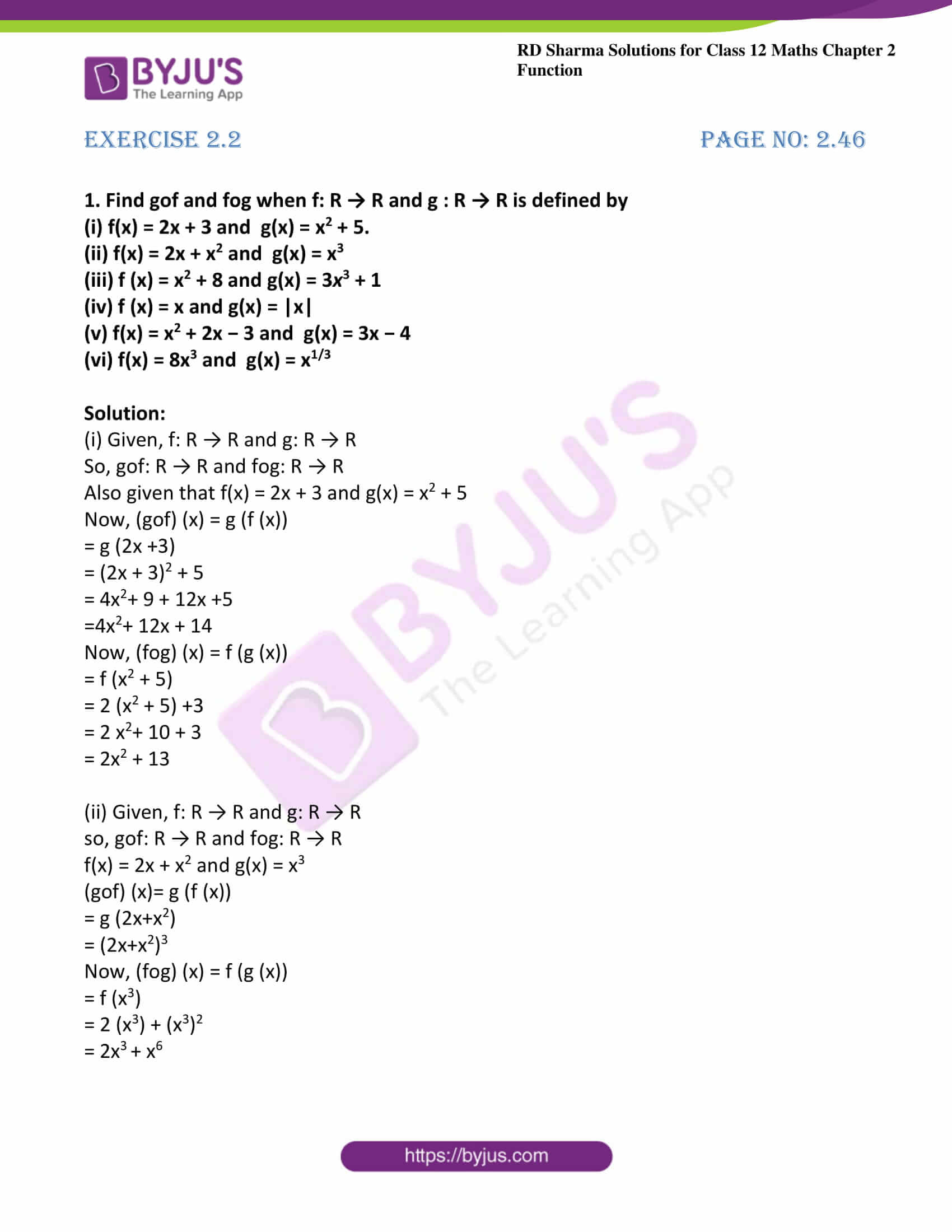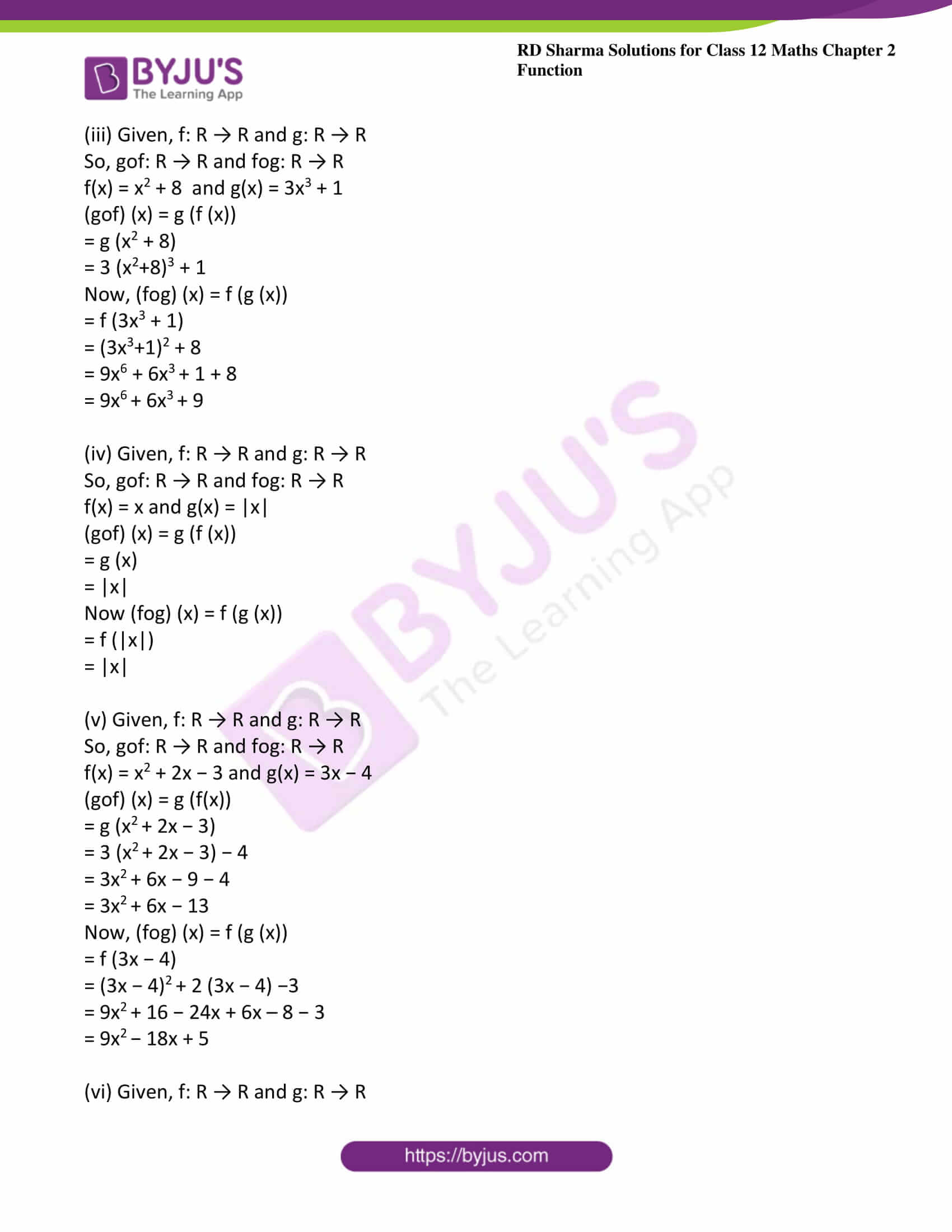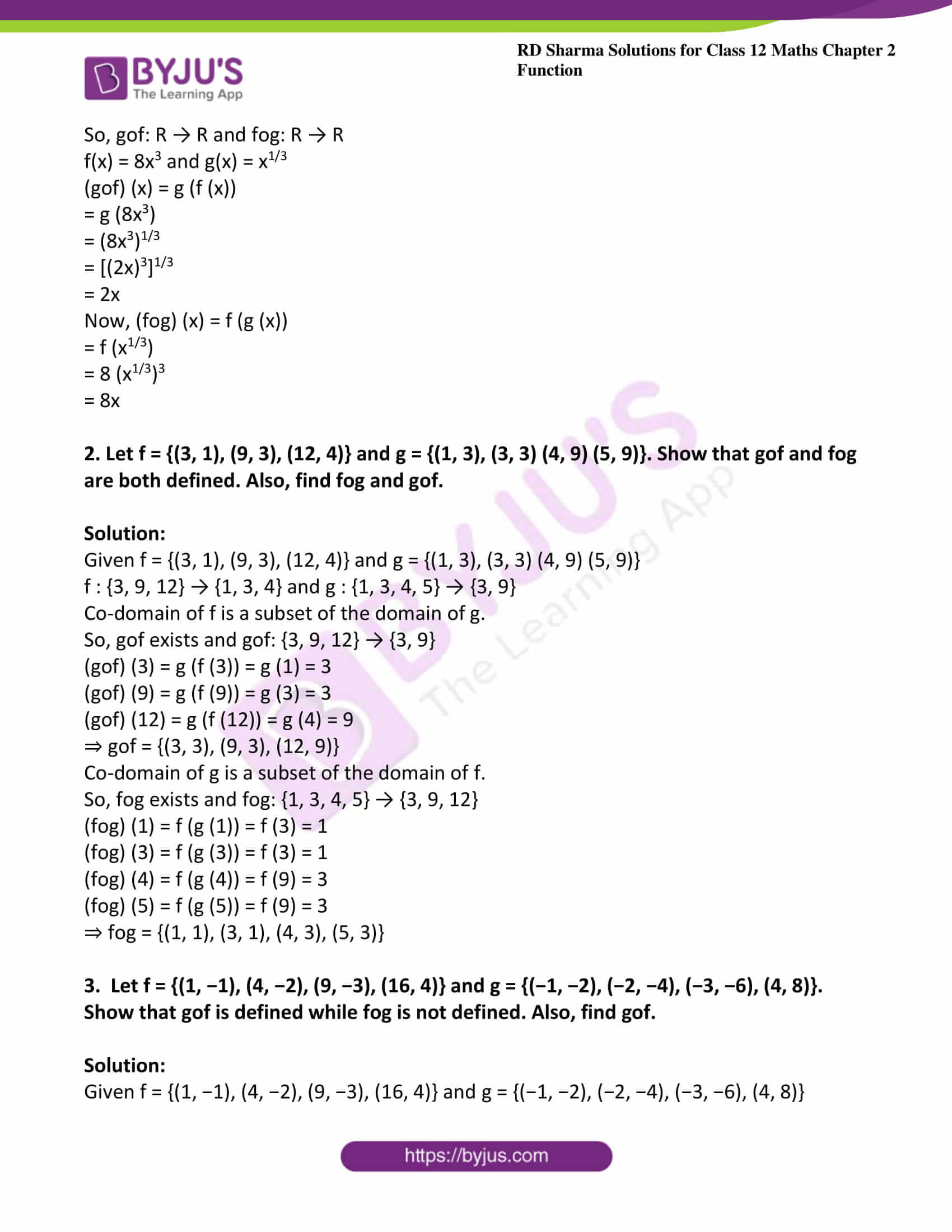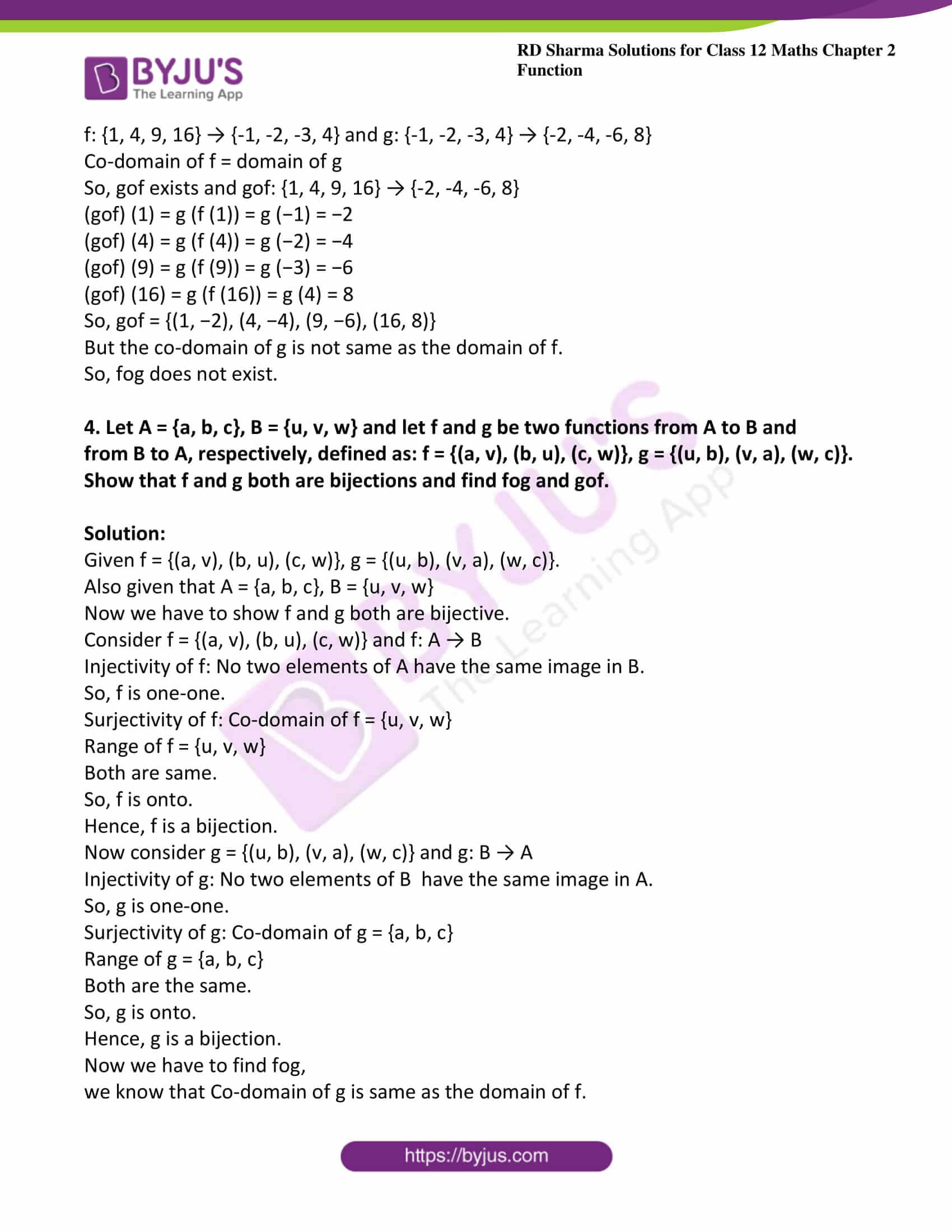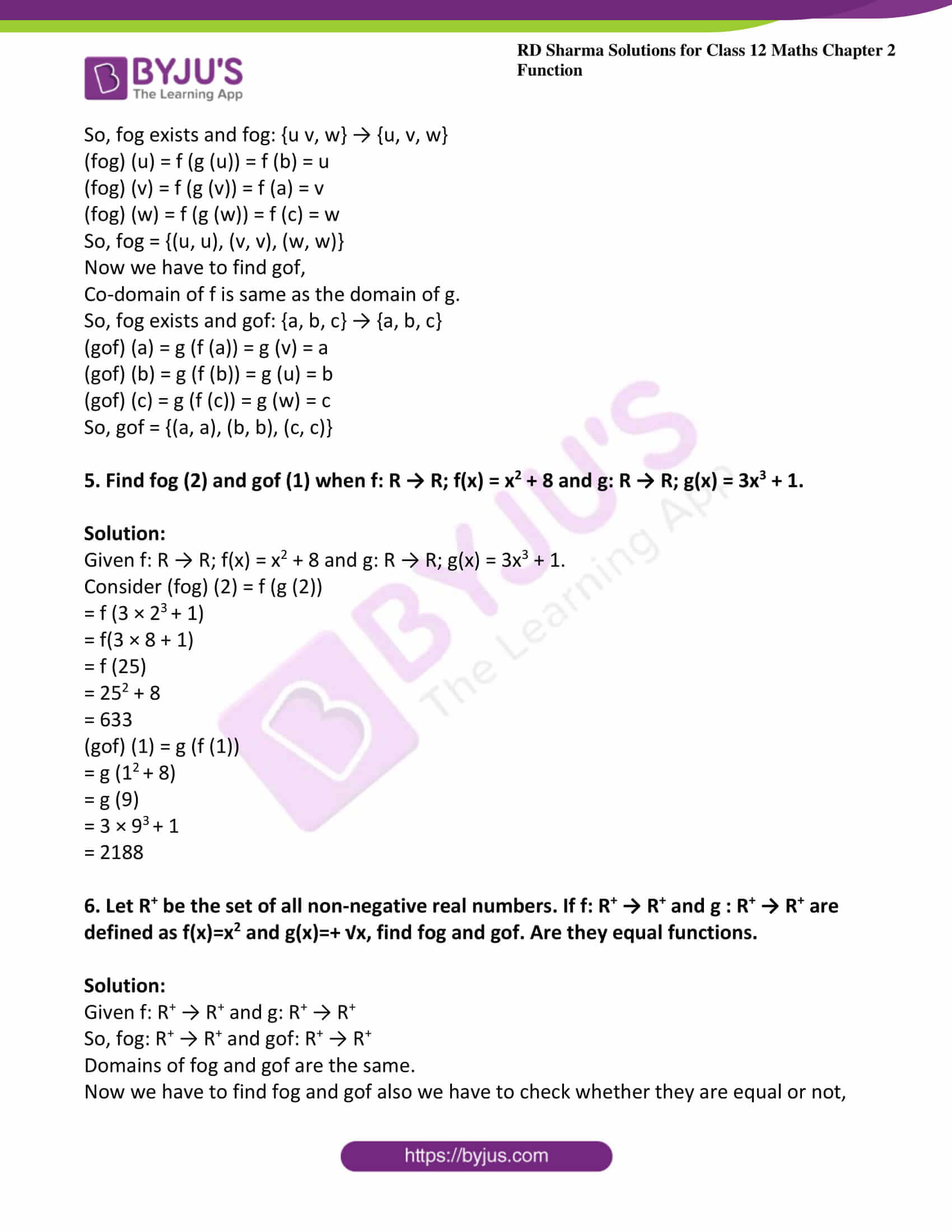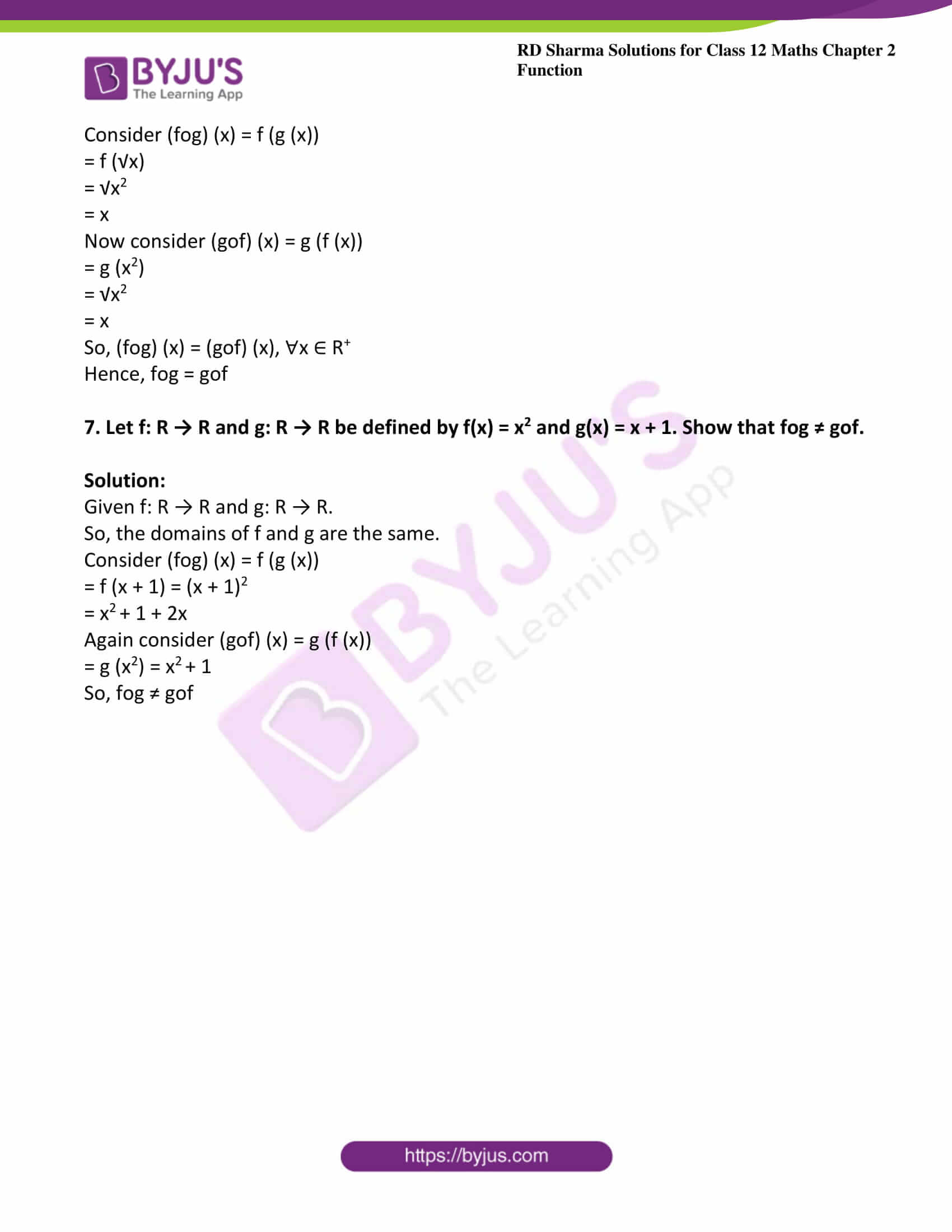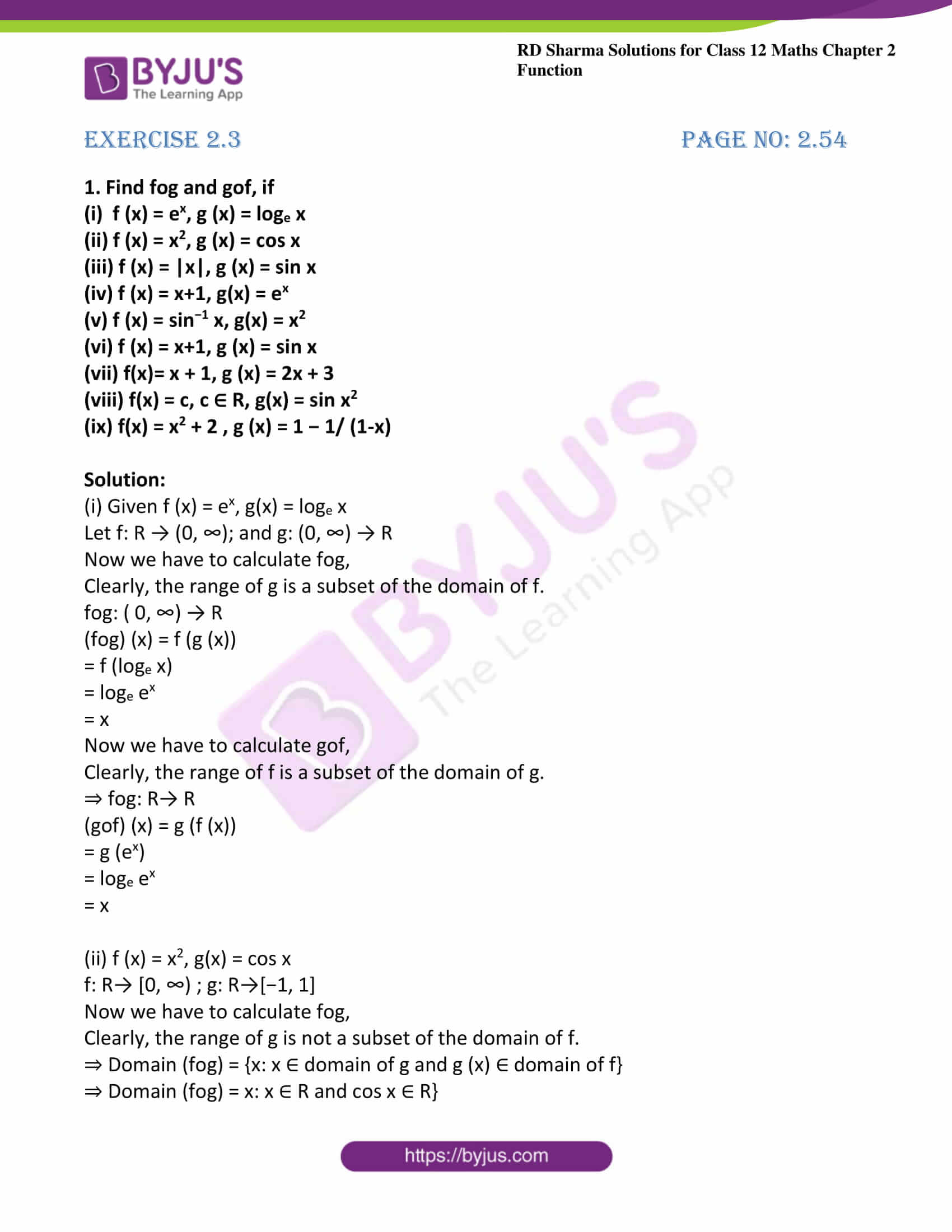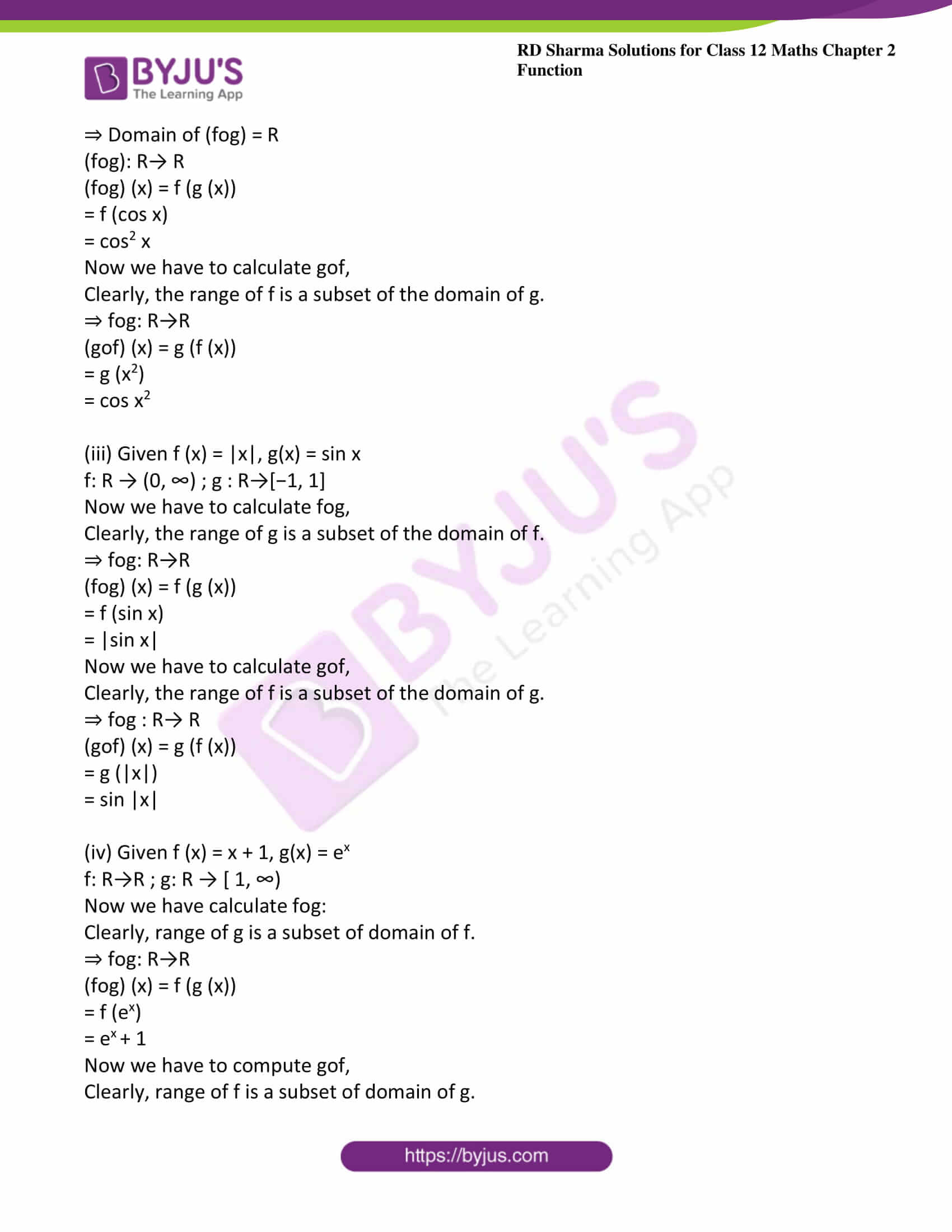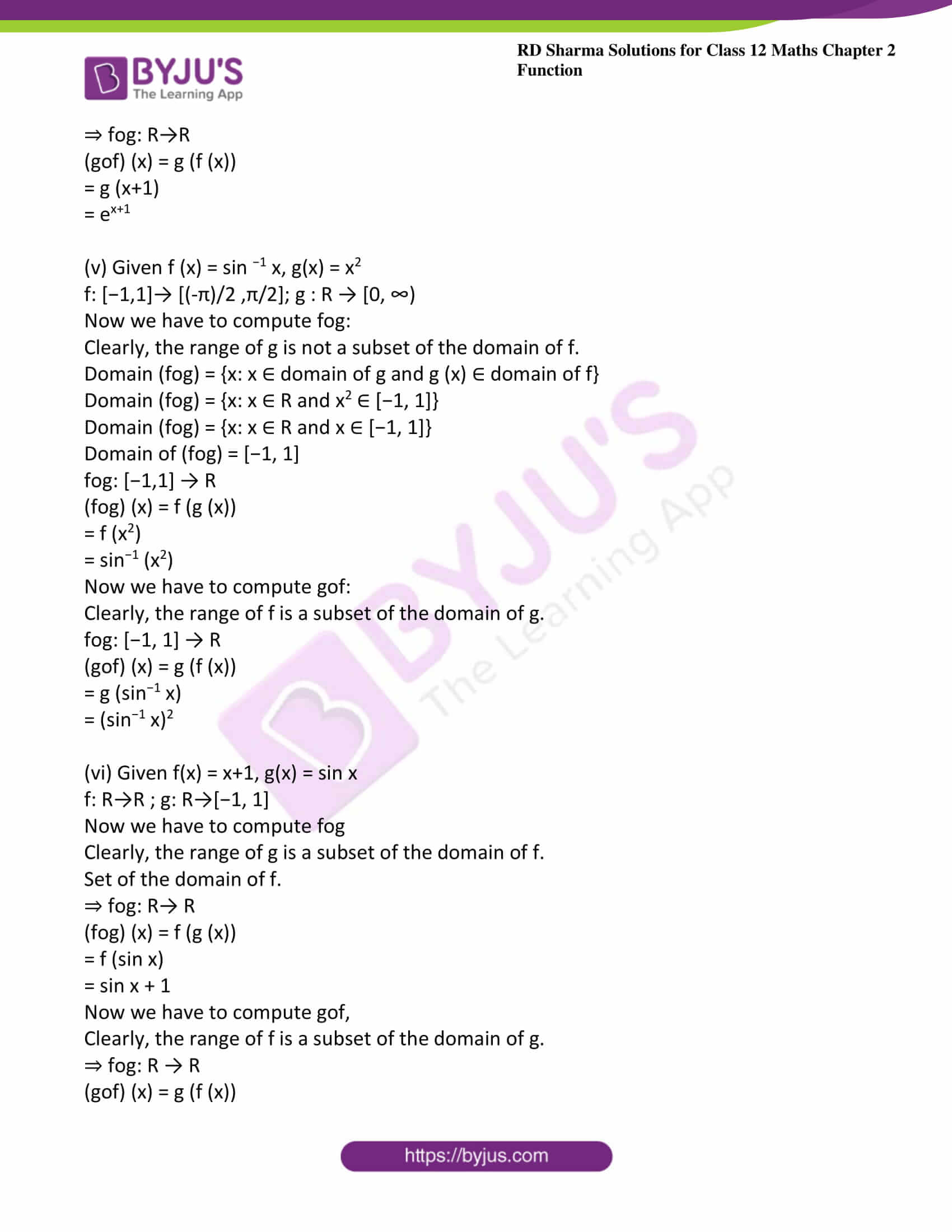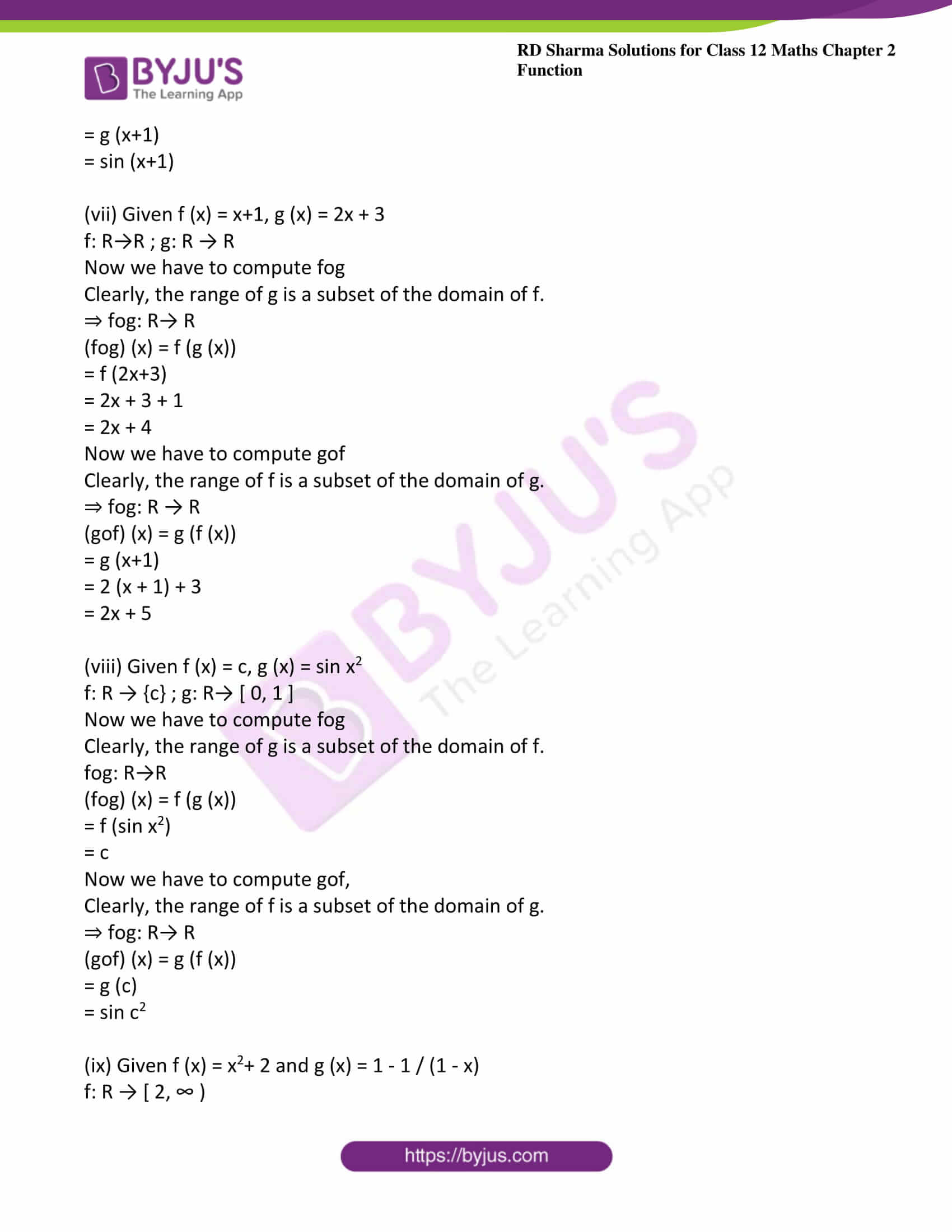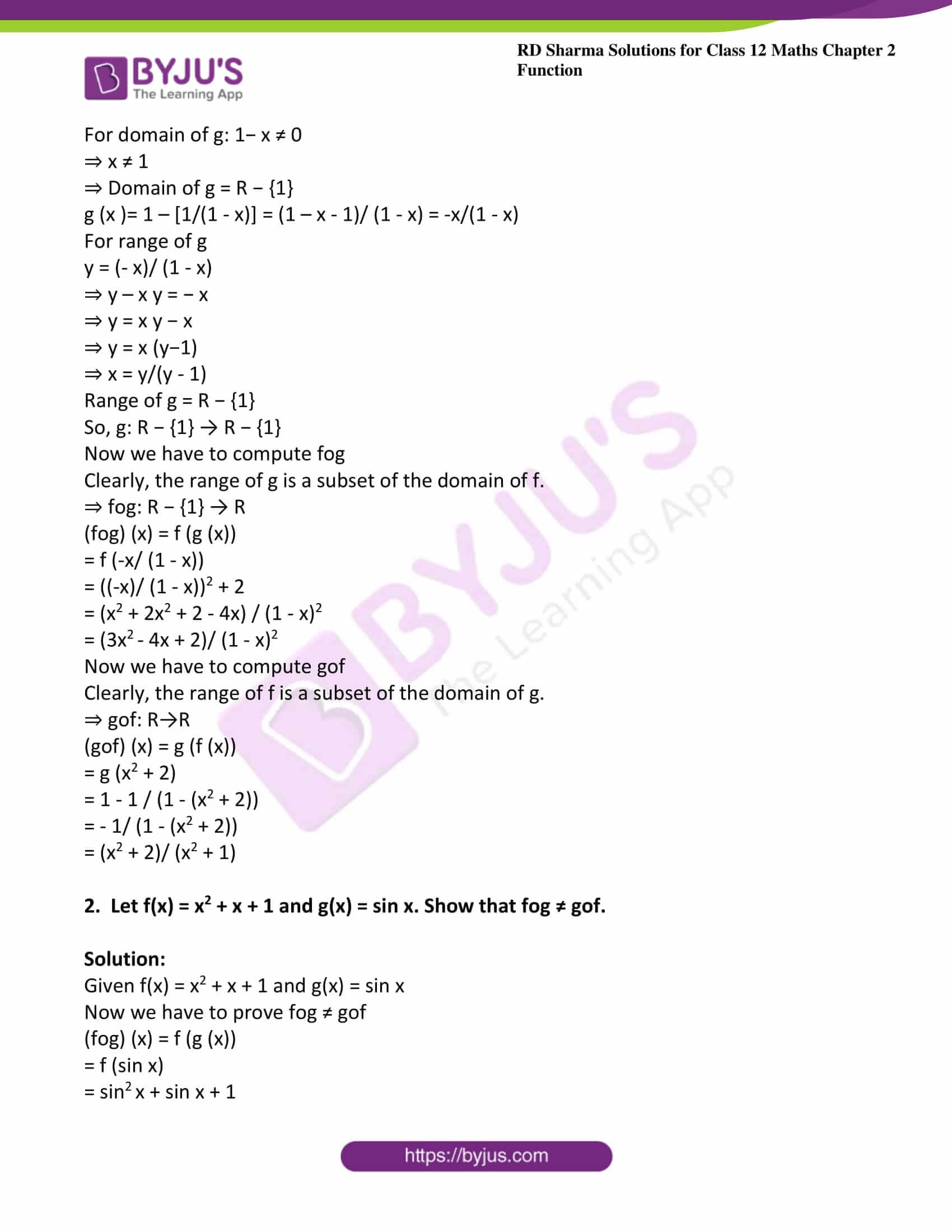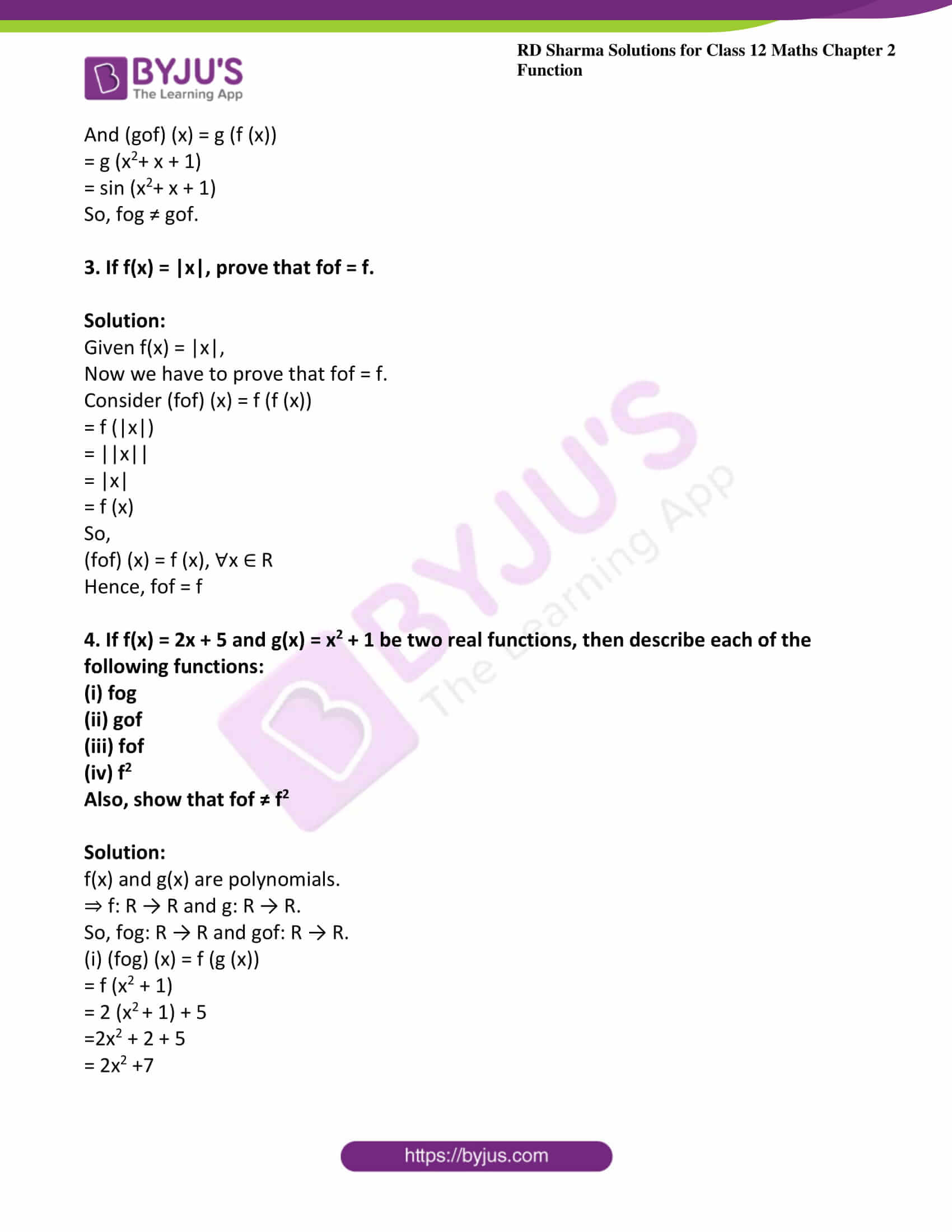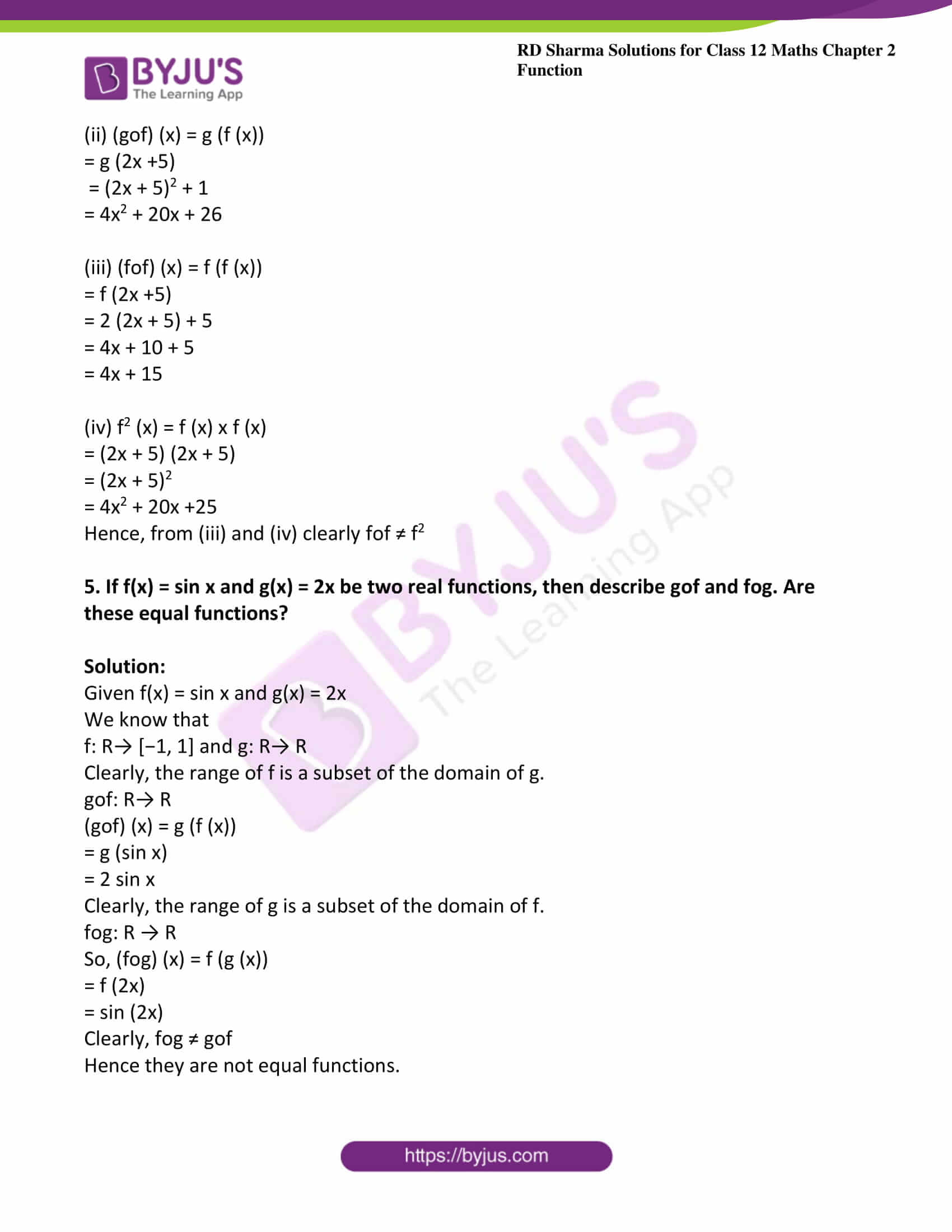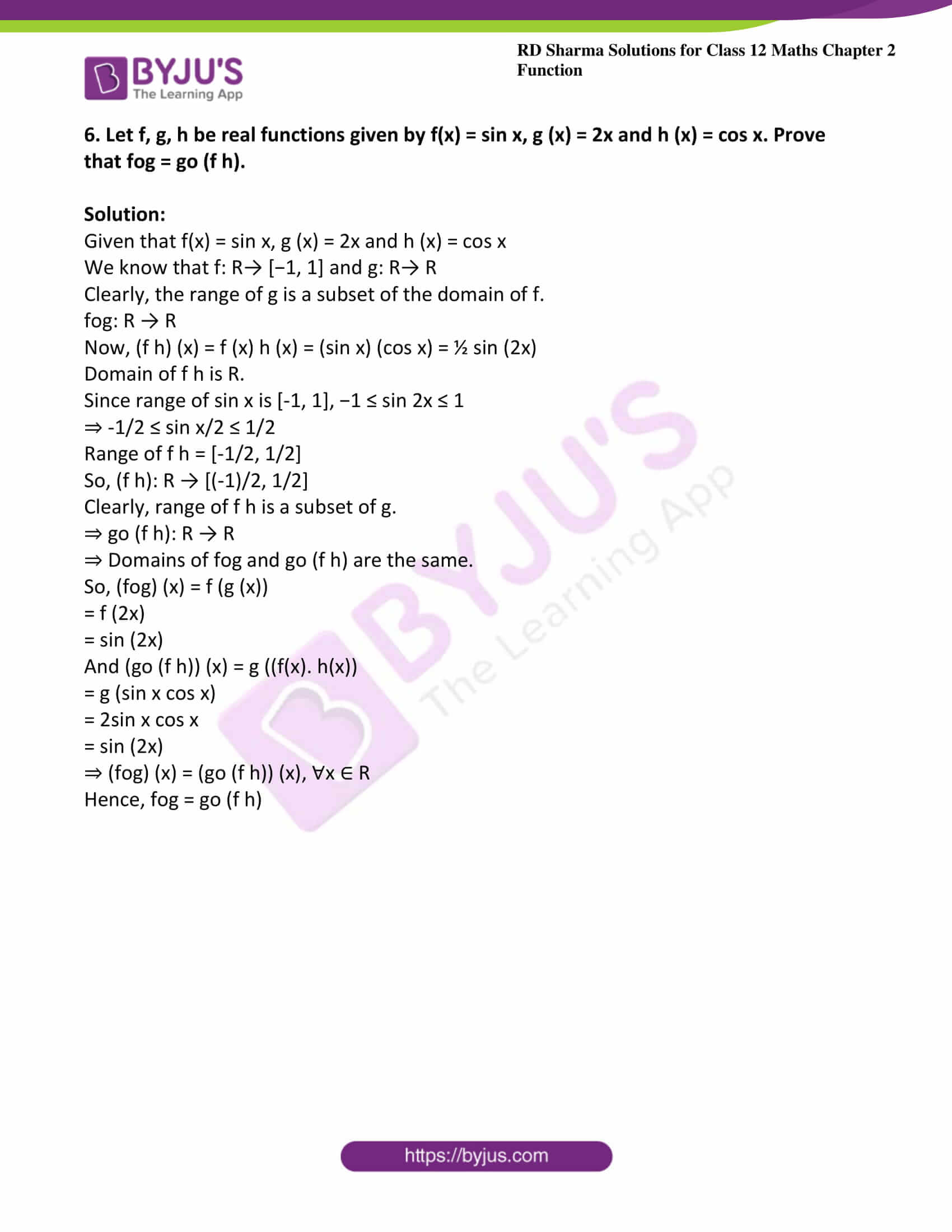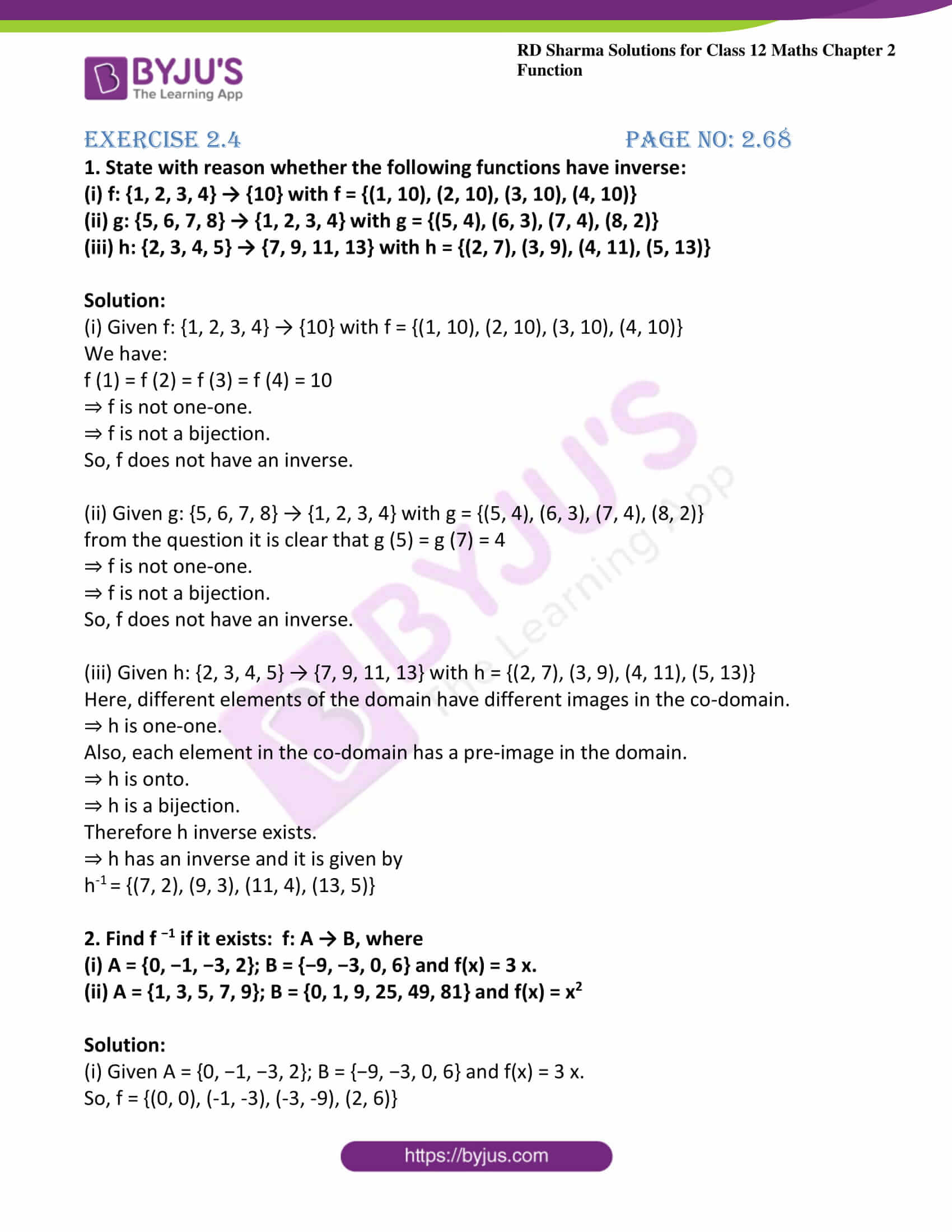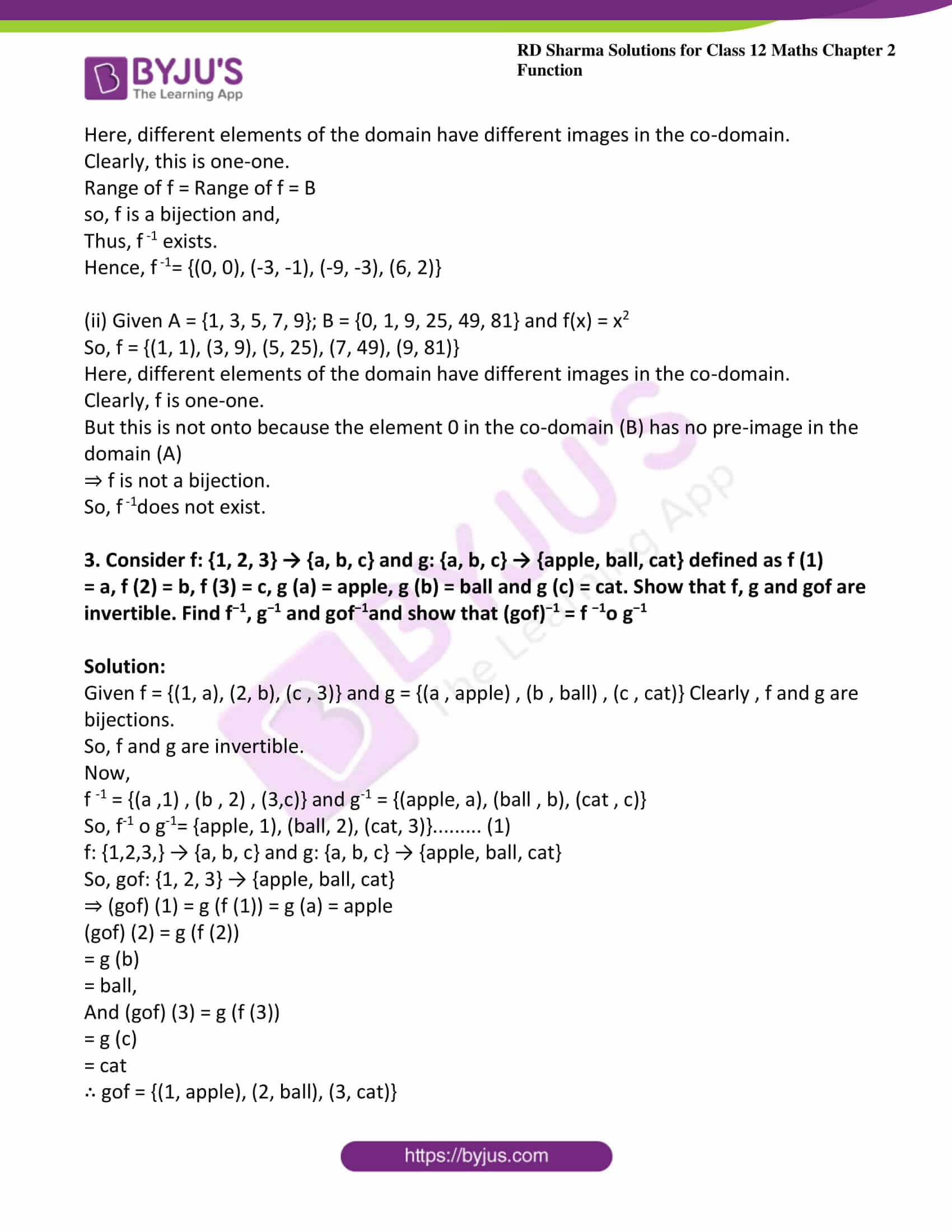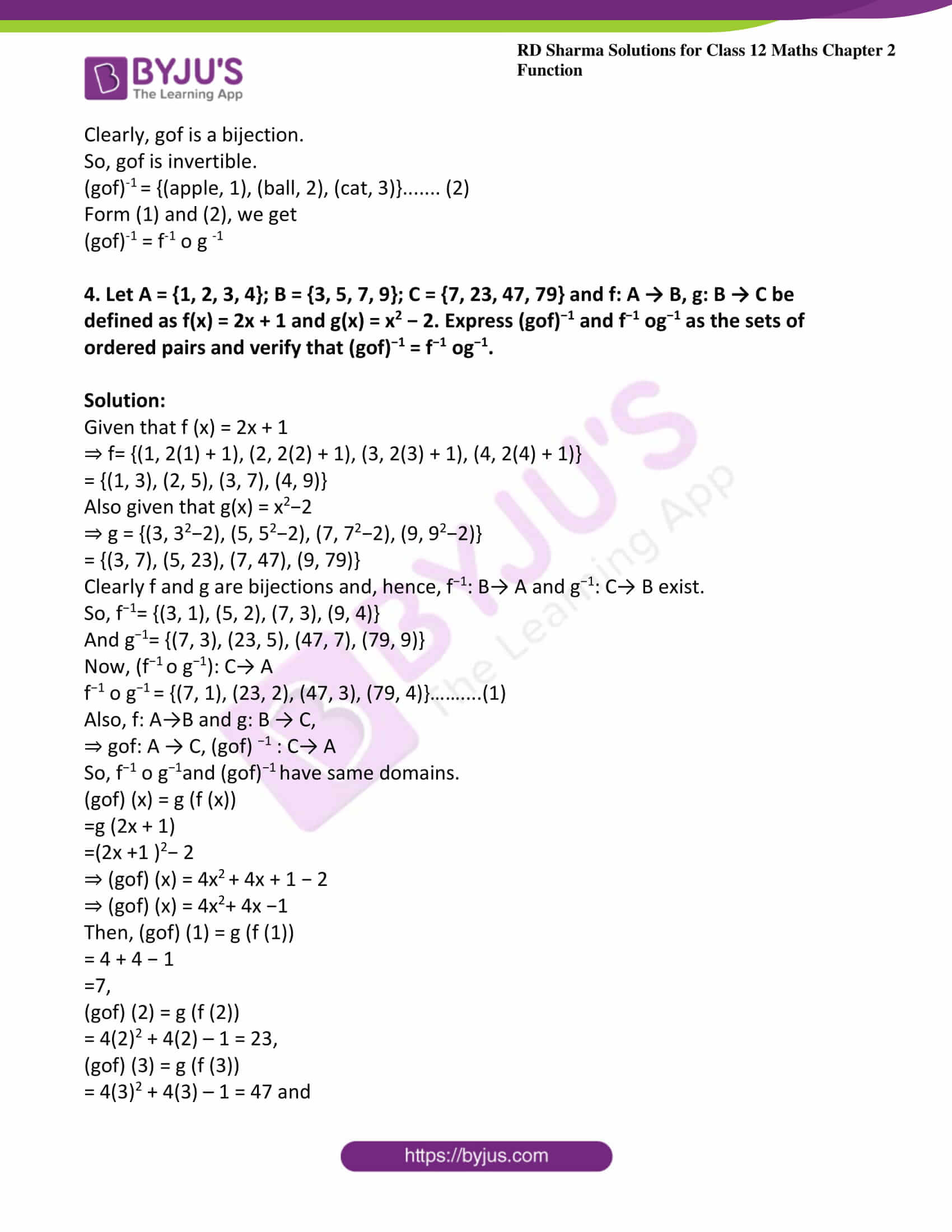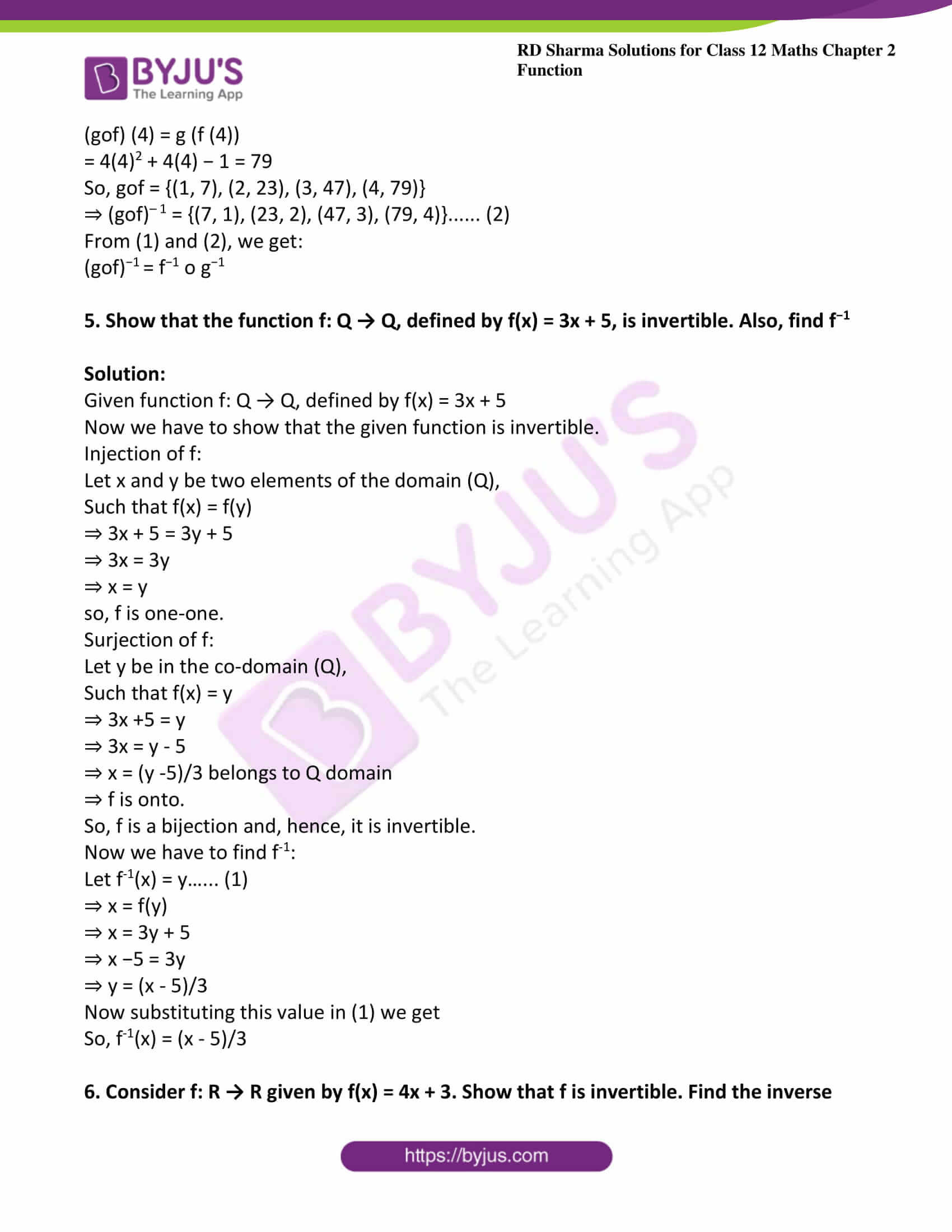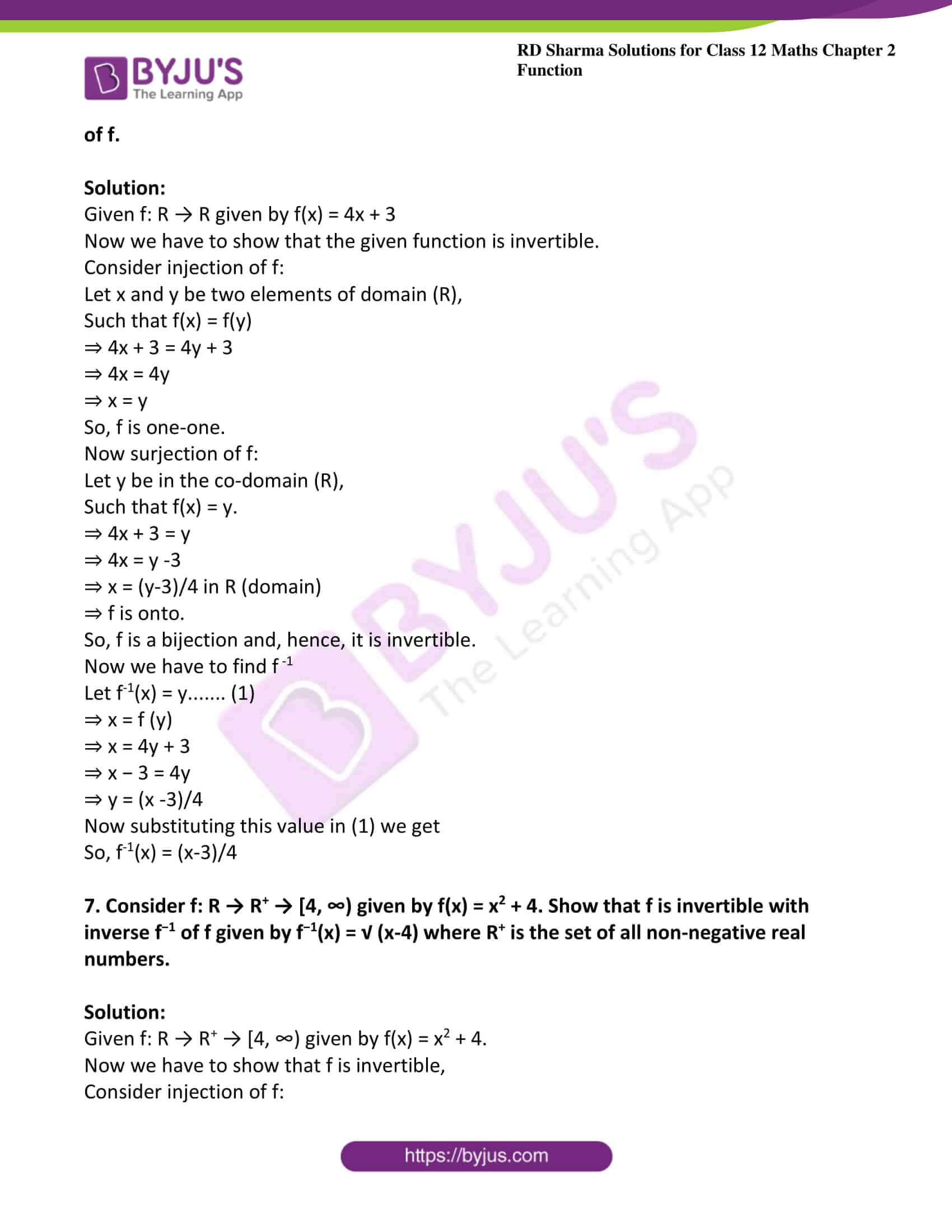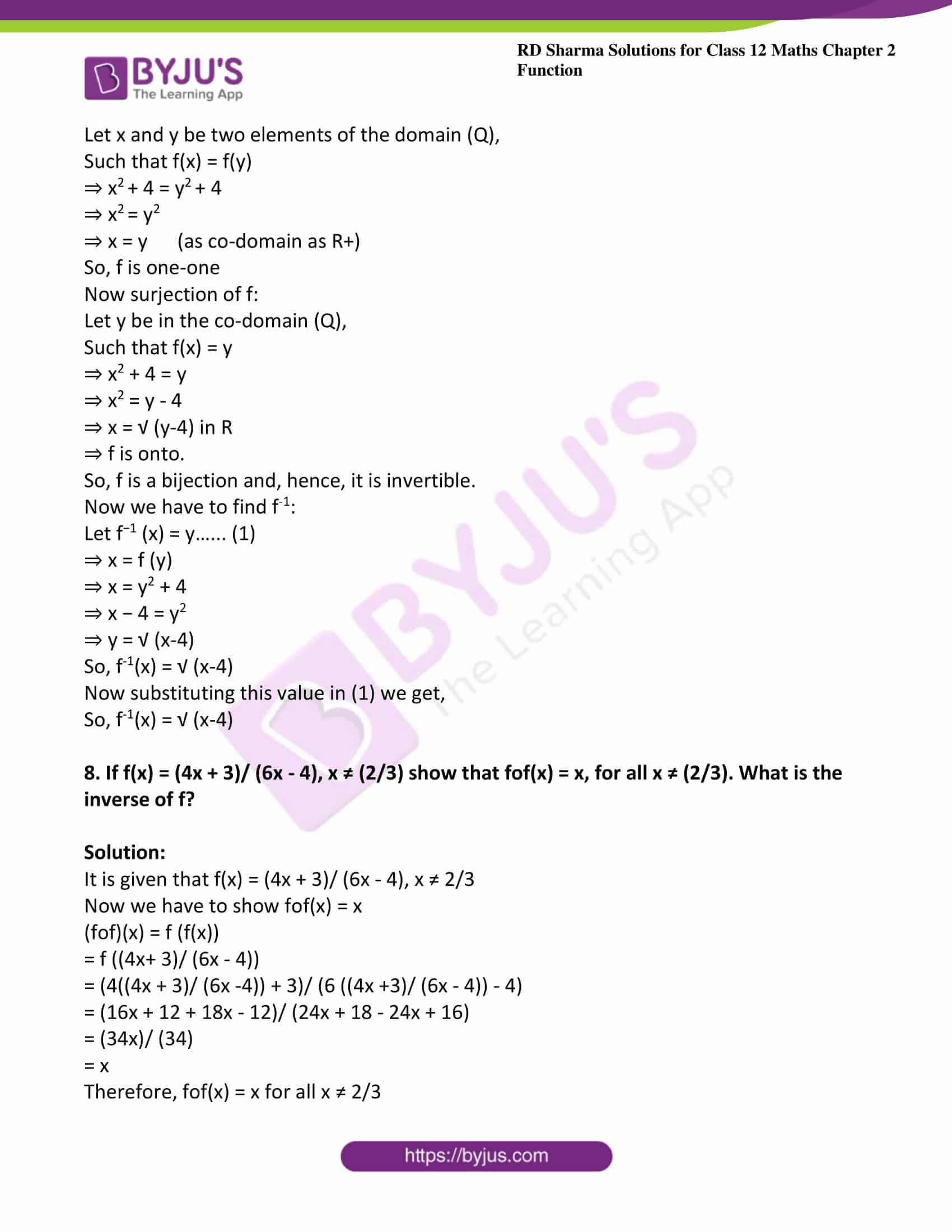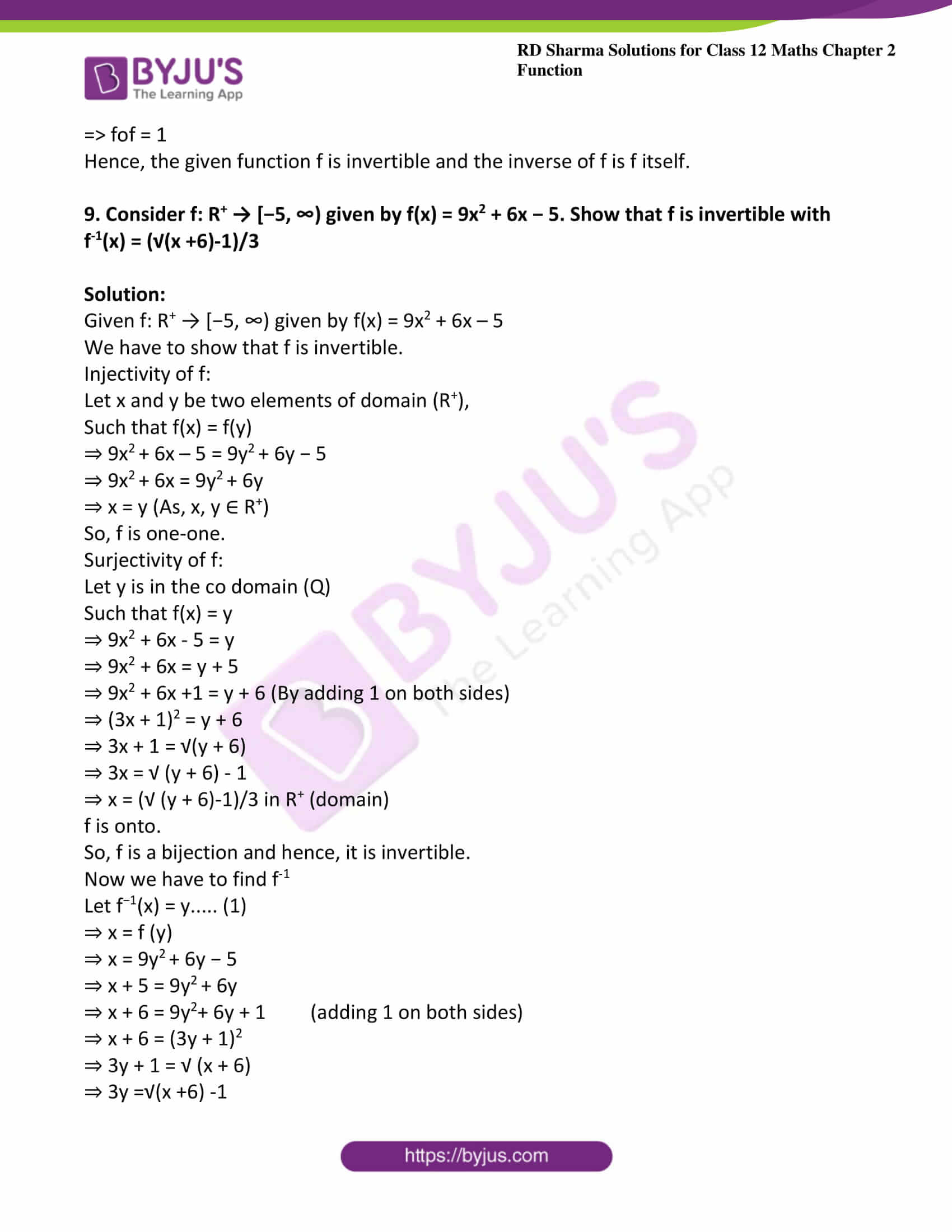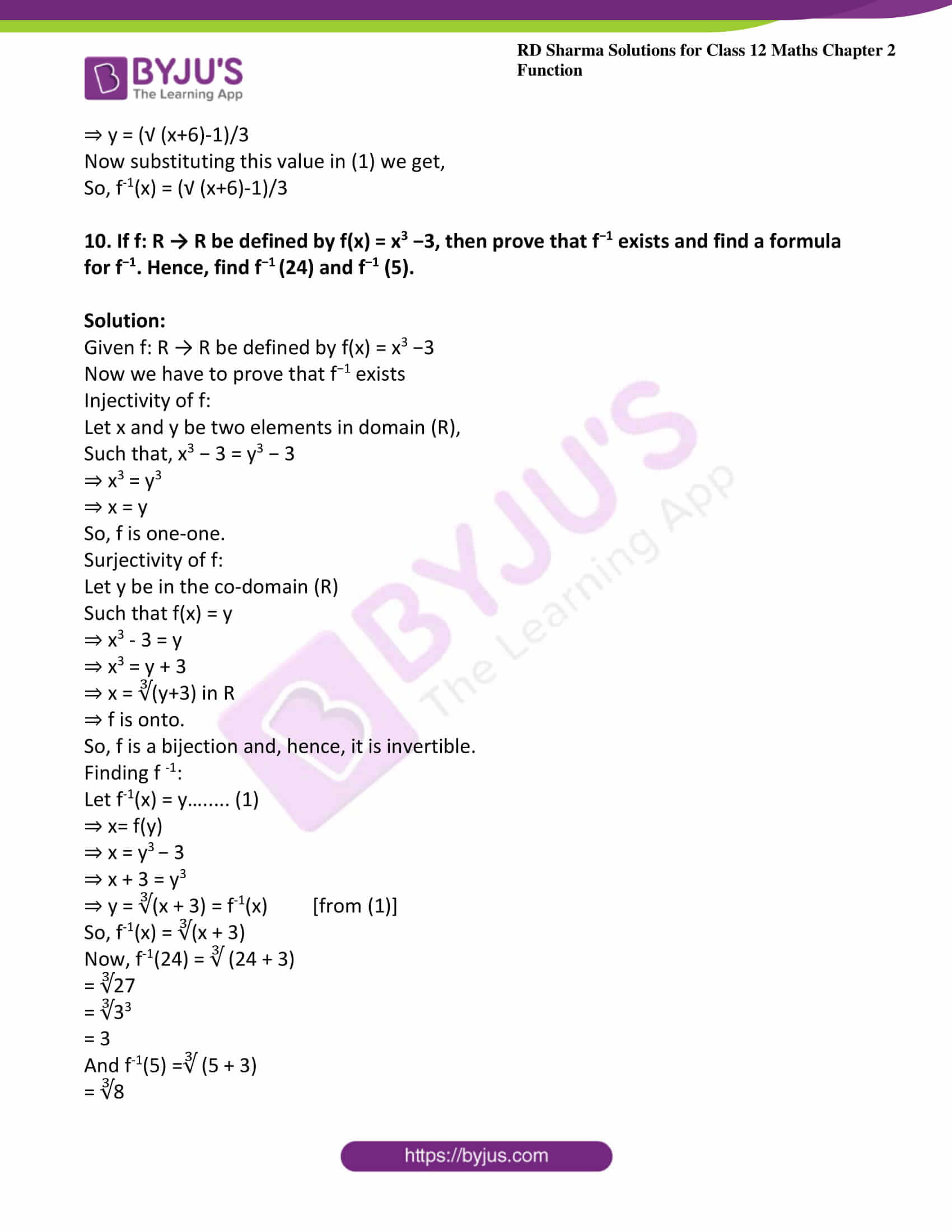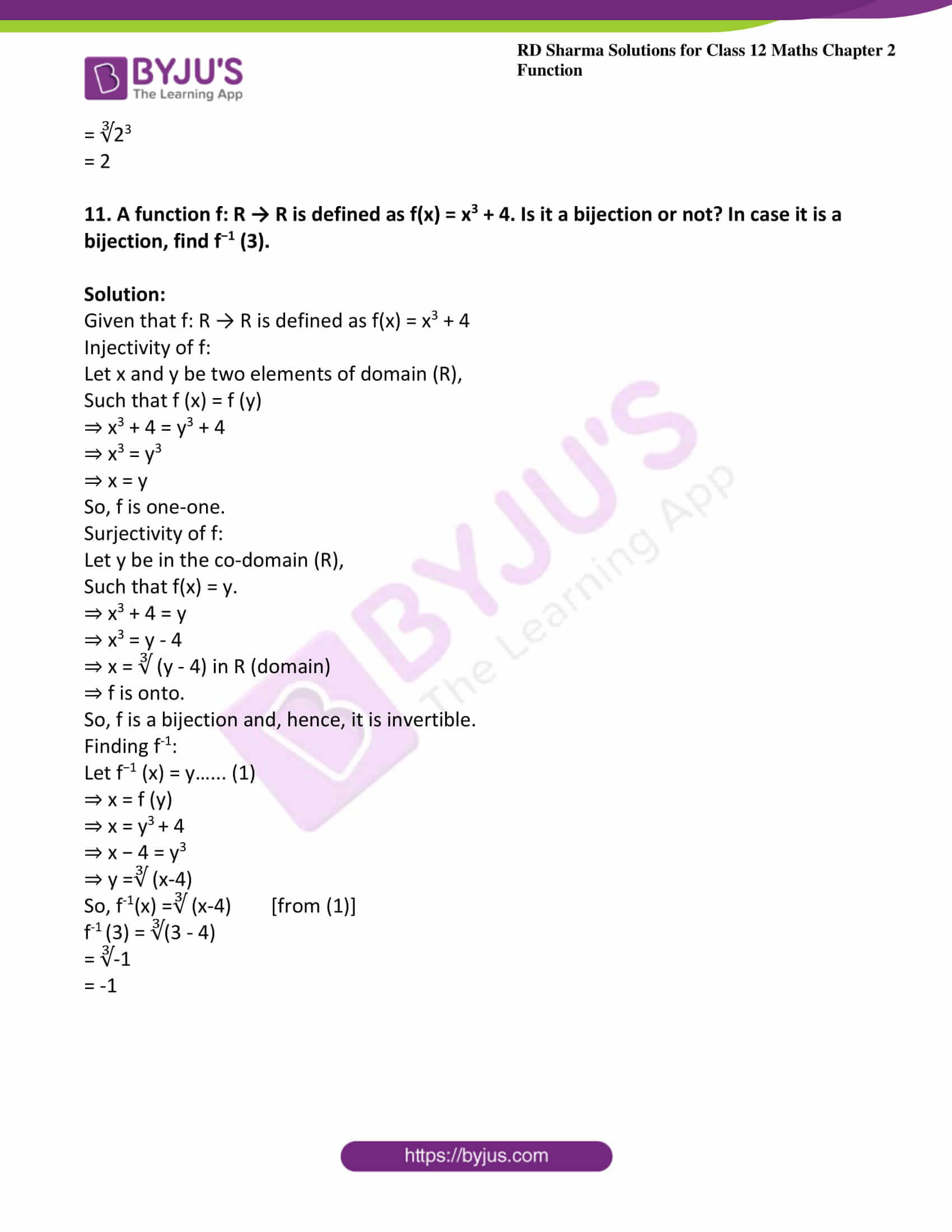### Exercise 2.1 Page No: 2.31

1. Give an example of a function

(i) Which is one-one but not onto.

(ii) Which is not one-one but onto.

(iii) Which is neither one-one nor onto.

Solution:

(i) Let f: Z → Z given by f(x) = 3x + 2

Let us check one-one condition on f(x) = 3x + 2

Injectivity:

Let x and y be any two elements in the domain (Z), such that f(x) = f(y).

f (x) = f(y)

⇒ 3x + 2 =3y + 2

⇒ 3x = 3y

⇒ x = y

⇒ f(x) = f(y)

⇒ x = y

So, f is one-one.

Surjectivity:

Let y be any element in the co-domain (Z), such that f(x) = y for some element x in Z(domain).

Let f(x) = y

⇒ 3x + 2 = y

⇒ 3x = y – 2

⇒ x = (y – 2)/3. It may not be in the domain (Z)

Because if we take y = 3,

x = (y – 2)/3 = (3-2)/3 = 1/3 ∉ domain Z.

So, for every element in the co domain there need not be any element in the domain such that f(x) = y.

Thus, f is not onto.

(ii) Example for the function which is not one-one but onto

Let f: Z → N ∪ {0} given by f(x) = |x|

Injectivity:

Let x and y be any two elements in the domain (Z),

Such that f(x) = f(y).

⇒ |x| = |y|

⇒ x = ± y

So, different elements of domain f may give the same image.

So, f is not one-one.

Surjectivity:

Let y be any element in the co domain (Z), such that f(x) = y for some element x in Z (domain).

f(x) = y

⇒ |x| = y

⇒ x = ± y

Which is an element in Z (domain).

So, for every element in the co-domain, there exists a pre-image in the domain.

Thus, f is onto.

(iii) Example for the function which is neither one-one nor onto.

Let f: Z → Z given by f(x) = 2x2 + 1

Injectivity:

Let x and y be any two elements in the domain (Z), such that f(x) = f(y).

f(x) = f(y)

⇒ 2x2+1 = 2y2+1

⇒ 2x2 = 2y2

⇒ x= y2

⇒ x = ± y

So, different elements of domain f may give the same image.

Thus, f is not one-one.

Surjectivity:

Let y be any element in the co-domain (Z), such that f(x) = y for some element x in Z (domain).

f (x) = y

⇒ 2x2+1=y

⇒ 2x2= y − 1

⇒ x2 = (y-1)/2

⇒ x = √ ((y-1)/2) ∉ Z always.

For example, if we take, y = 4,

x = ± √ ((y-1)/2)

= ± √ ((4-1)/2)

= ± √ (3/2) ∉ Z

So, x may not be in Z (domain).

Thus, f is not onto.

2. Which of the following functions from A to B are one-one and onto?
(i) f1 = {(1, 3), (2, 5), (3, 7)}; A = {1, 2, 3}, B = {3, 5, 7}

(ii) f2 = {(2, a), (3, b), (4, c)}; A = {2, 3, 4}, B = {a, b, c}

(iii) f3 = {(a, x), (b, x), (c, z), (d, z)}; A = {a, b, c, d,}, B = {x, y, z}.

Solution:

(i) Consider f1 = {(1, 3), (2, 5), (3, 7)}; A = {1, 2, 3}, B = {3, 5, 7}

Injectivity:

f1 (1) = 3

f(2) = 5

f1 (3) = 7

⇒ Every element of A has different images in B.

So, f1 is one-one.

Surjectivity:

Co-domain of f1 = {3, 5, 7}

Range of f1 =set of images  =  {3, 5, 7}

⇒ Co-domain = range

So, f1 is onto.

(ii) Consider f2 = {(2, a), (3, b), (4, c)}; A = {2, 3, 4}, B = {a, b, c}

Injectivity:

f2 (2) = a

f2 (3) = b

f2 (4) = c

⇒ Every element of A has different images in B.

So, f2 is one-one.

Surjectivity:

Co-domain of f2 = {a, b, c}

Range of f2 = set of images = {a, b, c}

⇒ Co-domain = range

So, f2 is onto.

(iii) Consider f3 = {(a, x), (b, x), (c, z), (d, z)} ; A = {a, b, c, d,}, B = {x, y, z}

Injectivity:

f3 (a) = x

f3 (b) = x

f3 (c) = z

f3 (d) = z

⇒ a and b have the same image x.

Also c and d have the same image z

So, f3 is not one-one.

Surjectivity:

Co-domain of f3 ={x, y, z}

Range of f3 =set of images = {x, z}

So, the co-domain  is not same as the range.

So, f3 is not onto.

3. Prove that the function f: N → N, defined by f(x) = x2 + x + 1, is one-one but not onto

Solution:

Given f: N → N, defined by f(x) = x2 + x + 1

Now we have to prove that given function is one-one

Injectivity:

Let x and y be any two elements in the domain (N), such that f(x) = f(y).

⇒ x2 + x + 1 = y2 + y + 1

⇒ (x2 – y2) + (x – y) = 0 

⇒ (x + y) (x- y ) + (x – y ) = 0

⇒ (x – y) (x + y + 1) = 0

⇒ x – y = 0 [x + y + 1 cannot be zero because x and y are natural numbers

⇒ x = y

So, f is one-one.

Surjectivity:

When x = 1

x2 + x + 1 = 1 + 1 + 1 = 3

⇒ x2 + x +1 ≥ 3, for every x in N.

⇒ f(x) will not assume the values 1 and 2.

So, f is not onto.

4. Let A = {−1, 0, 1} and f = {(x, x2) : x ∈ A}. Show that f : A → A is neither one-one nor onto.

Solution:

Given A = {−1, 0, 1} and f = {(x, x2): x ∈ A}

Also given that, f(x) = x2

Now we have to prove that given function neither one-one or nor onto.

Injectivity:

Let x = 1

Therefore f(1) = 12=1 and

f(-1)=(-1)2=1

⇒ 1 and -1 have the same images.

So, f is not one-one.

Surjectivity:

Co-domain of f = {-1, 0, 1}

f(1) = 12 = 1,

f(-1) = (-1)2 = 1 and

f(0) = 0

⇒ Range of f  = {0, 1}

So, both are not same.

Hence, f is not onto

5. Classify the following function as injection, surjection or bijection:

(i) f: N → N given by f(x) = x2

(ii) f: Z → Z given by f(x) = x2

(iii) f: N → N given by f(x) = x3

(iv) f: Z → Z given by f(x) = x3

(v) f: R → R, defined by f(x) = |x|

(vi) f: Z → Z, defined by f(x) = x2 + x

(vii) f: Z → Z, defined by f(x) = x − 5

(viii) f: R → R, defined by f(x) = sin x

(ix) f: R → R, defined by f(x) = x3 + 1

(x) f: R → R, defined by f(x) = x3 − x

(xi) f: R → R, defined by f(x) = sin2x + cos2x

(xii) f: Q − {3} → Q, defined by f (x) = (2x +3)/(x-3)

(xiii) f: Q → Q, defined by f(x) = x3 + 1

(xiv) f: R → R, defined by f(x) = 5x3 + 4

(xv) f: R → R, defined by f(x) = 5x3 + 4

(xvi) f: R → R, defined by f(x) = 1 + x2

(xvii) f: R → R, defined by f(x) = x/(x2 + 1)

Solution:

(i) Given f: N → N, given by f(x) = x2

Now we have to check for the given function is injection, surjection and bijection condition.

Injection condition:

Let x and y be any two elements in the domain (N), such that f(x) = f(y).

f(x) = f(y)

x2 = y2

x = y (We do not get ± because x and y are in N that is natural numbers)

So, f is an injection.

Surjection condition:

Let y be any element in the co-domain (N), such that f(x) = y for some element x in N (domain).

f(x) = y

x2= y

x = √y, which may not be in N.

For example, if y = 3,

x = √3 is not in N.

So, f is not a surjection.

Also f is not a bijection.

(ii) Given f: Z → Z, given by f(x) = x2

Now we have to check for the given function is injection, surjection and bijection condition.

Injection condition:

Let x and y be any two elements in the domain (Z), such that f(x) = f(y).

f(x) = f(y)

x2 = y2

x = ±y

So, f is not an injection.

Surjection test:

Let y be any element in the co-domain (Z), such that f(x) = y for some element x in Z (domain).

f(x) = y

x2 = y

x = ± √y which may not be in Z.

For example, if y = 3,

x = ± √ 3 is not in Z.

So, f is not a surjection.

Also f is not bijection.

(iii) Given f: N → N given by f(x) = x3

Now we have to check for the given function is injection, surjection and bijection condition.

Injection condition:

Let x and y be any two elements in the domain (N), such that f(x) = f(y).

f(x) = f(y)

x3 = y3

x = y

So, f is an injection

Surjection condition:

Let y be any element in the co-domain (N), such that f(x) = y for some element x in N (domain).

f(x) = y

x3= y

x = ∛y which may not be in N.

For example, if y = 3,

X = ∛3 is not in N.

So, f is not a surjection and f is not a bijection.

(iv) Given f: Z → Z given by f(x) = x3

Now we have to check for the given function is injection, surjection and bijection condition.

Injection condition:

Let x and y be any two elements in the domain (Z), such that f(x) = f(y)

f(x) = f(y)

x3 = y3

x = y

So, f is an injection.

Surjection condition:

Let y be any element in the co-domain (Z), such that f(x) = y for some element x in Z (domain).

f(x) = y

x3 = y

x = ∛y which may not be in Z.

For example, if y = 3,

x = ∛3 is not in Z.

So, f is not a surjection and f is not a bijection.

(v) Given f: R → R, defined by f(x) = |x|

Now we have to check for the given function is injection, surjection and bijection condition.

Injection test:

Let x and y be any two elements in the domain (R), such that f(x) = f(y)

f(x) = f(y)

|x|=|y|

x = ±y

So, f is not an injection.

Surjection test:

Let y be any element in the co-domain (R), such that f(x) = y for some element x in R (domain).

f(x) = y

|x|=y

x = ± y ∈ Z

So, f is a surjection and f is not a bijection.

(vi) Given f: Z → Z, defined by f(x) = x2 + x

Now we have to check for the given function is injection, surjection and bijection condition.

Injection test:

Let x and y be any two elements in the domain (Z), such that f(x) = f(y).

f(x) = f(y)

x2+ x = y+ y

Here, we cannot say that x = y.

For example, x = 2 and y = – 3

Then,

x2 + x = 22 + 2 = 6

y2 + y = (−3)2 – 3 = 6

So, we have two numbers 2 and -3 in the domain Z whose image is same as 6.

So, f is not an injection.

Surjection test:

Let y be any element in the co-domain (Z),

such that f(x) = y for some element x in Z (domain).

f(x) = y

x2 + x = y

Here, we cannot say x ∈ Z.

For example, y = – 4.

x2 + x = − 4

x2 + x + 4 = 0

x = (-1 ± √-5)/2 = (-1 ± i √5)/2 which is not in Z.

So, f is not a surjection and f is not a bijection.

(vii) Given f: Z → Z, defined by f(x) = x – 5

Now we have to check for the given function is injection, surjection and bijection condition.

Injection test:

Let x and y be any two elements in the domain (Z), such that f(x) = f(y).

f(x) = f(y)

x – 5 = y – 5

x = y

So, f is an injection.

Surjection test:

Let y be any element in the co-domain (Z), such that f(x) = y for some element x in Z (domain).

f(x) = y

x – 5 = y

x = y + 5, which is in Z.

So, f is a surjection and f is a bijection

(viii) Given f: R → R, defined by f(x) = sin x

Now we have to check for the given function is injection, surjection and bijection condition.

Injection test:

Let x and y be any two elements in the domain (R), such that f(x) = f(y).

f(x) = f(y)

Sin x = sin y

Here, x may not be equal to y because sin 0 = sin π.

So, 0 and π have the same image 0.

So, f is not an injection.

Surjection test:

Range of f = [-1, 1]

Co-domain of f = R

Both are not same.

So, f is not a surjection and f is not a bijection.

(ix) Given f: R → R, defined by f(x) = x3 + 1

Now we have to check for the given function is injection, surjection and bijection condition.

Injection test:

Let x and y be any two elements in the domain (R), such that f(x) = f(y).

f(x) = f(y)

x3+1 = y3+ 1

x3= y3

x = y

So, f is an injection.

Surjection test:

Let y be any element in the co-domain (R), such that f(x) = y for some element x in R (domain).

f(x) = y

x3+1=y

x = ∛ (y – 1) ∈ R

So, f is a surjection.

So, f is a bijection.

(x)  Given f: R → R, defined by f(x) = x3 − x

Now we have to check for the given function is injection, surjection and bijection condition.

Injection test:

Let x and y be any two elements in the domain (R), such that f(x) = f(y).

f(x) = f(y)

x3 – x = y3 − y

Here, we cannot say x = y.

For example, x = 1 and y = -1

x3 − x = 1 − 1 = 0

y3 – y = (−1)3− (−1) – 1 + 1 = 0

So, 1 and -1 have the same image 0.

So, f is not an injection.

Surjection test:

Let y be any element in the co-domain (R), such that f(x) = y for some element x in R (domain).

f(x) = y

x3 − x = y

By observation we can say that there exist some x in R, such that x– x = y.

So, f is a surjection and f is not a bijection.

(xi) Given f: R → R, defined by f(x) = sin2x + cos2x

Now we have to check for the given function is injection, surjection and bijection condition.

Injection condition:

f(x) = sin2x + cos2x

We know that sin2x + cos2x = 1

So, f(x) = 1 for every x in R.

So, for all elements in the domain, the image is 1.

So, f is not an injection.

Surjection condition:

Range of f = {1}

Co-domain of f = R

Both are not same.

So, f is not a surjection and f is not a bijection.

(xii) Given f: Q − {3} → Q, defined by f (x) = (2x +3)/(x-3)

Now we have to check for the given function is injection, surjection and bijection condition.

Injection test:

Let x and y be any two elements in the domain (Q − {3}), such that f(x) = f(y).

f(x) = f(y)

(2x + 3)/(x – 3) = (2y + 3)/(y – 3)

(2x + 3) (y − 3) = (2y + 3) (x − 3)

2xy − 6x + 3y − 9 = 2xy − 6y + 3x − 9

9x = 9y

x = y

So, f is an injection.

Surjection test:

Let y be any element in the co-domain (Q − {3}), such that f(x) = y for some element x in Q (domain).

f(x) = y

(2x + 3)/(x – 3) = y

2x + 3 = x y − 3y

2x – x y = −3y − 3

x (2−y) = −3 (y + 1)

x = -3(y + 1)/(2 – y) which is not defined at y = 2.

So, f is not a surjection and f is not a bijection.

(xiii) Given f: Q → Q, defined by f(x) = x3 + 1

Now we have to check for the given function is injection, surjection and bijection condition.

Injection test:

Let x and y be any two elements in the domain (Q), such that f(x) = f(y).

f(x) = f(y)

x3 + 1 = y3 + 1

x3 = y3

x = y

So, f is an injection.

Surjection test:

Let y be any element in the co-domain (Q), such that f(x) = y for some element x in Q (domain).

f(x) = y

x3+ 1 = y

x = ∛(y-1), which may not be in Q.

For example, if y= 8,

x3+ 1 =  8

x3= 7

x = ∛7, which is not in Q.

So, f is not a surjection and f is not a bijection.

(xiv) Given f: R → R, defined by f(x) = 5x3 + 4

Now we have to check for the given function is injection, surjection and bijection condition.

Injection test:

Let x and y be any two elements in the domain (R), such that f(x) = f(y).

f(x) = f(y)

5x3 + 4 = 5y3 + 4

5x3= 5y3

x3 = y3

x = y

So, f is an injection.

Surjection test:

Let y be any element in the co-domain (R), such that f(x) = y for some element x in R (domain).

f(x) = y

5x3+ 4 = y

x3 = (y – 4)/5 ∈ R

So, f is a surjection and f is a bijection.

(xv) Given f: R → R, defined by f(x) = 5x3 + 4

Now we have to check for the given function is injection, surjection and bijection condition.

Injection condition:

Let x and y be any two elements in the domain (R), such that f(x) = f(y).

f(x) = f(y)

5x3 + 4 = 5y3 + 4

5x3 = 5y3

x3 = y3

x = y

So, f is an injection.

Surjection test:

Let y be any element in the co-domain (R), such that f(x) = y for some element x in R (domain).

f(x) = y

5x3 + 4 = y

x3 = (y – 4)/5 ∈ R

So, f is a surjection and f is a bijection.

(xvi) Given f: R → R, defined by f(x) = 1 + x2

Now we have to check for the given function is injection, surjection and bijection condition.

Injection condition:

Let x and y be any two elements in the domain (R), such that f(x) = f(y).

f(x) = f(y)

1 + x2 = 1 + y2

x= y2

x = ± y

So, f is not an injection.

Surjection test:

Let y be any element in the co-domain (R), such that f(x) = y for some element x in R (domain).

f(x) = y

1 + x2 = y

x2 = y − 1

x = ± √-1 = ± i is not in R.

So, f is not a surjection and f is not a bijection.

(xvii) Given f: R → R, defined by f(x) = x/(x2 + 1)

Now we have to check for the given function is injection, surjection and bijection condition.

Injection condition:

Let x and y be any two elements in the domain (R), such that f(x) = f(y).

f(x) = f(y)

x /(x2 + 1) = y /(y2 + 1)

x y2+ x = x2y + y

xy2 − x2y + x − y = 0

−x y (−y + x) + 1 (x − y) = 0

(x − y) (1 – x y) = 0

x = y or x = 1/y

So, f is not an injection.

Surjection test:

Let y be any element in the co-domain (R), such that f(x) = y for some element x in R (domain).

f(x) = y

x /(x2 + 1) = y

y x2 – x + y = 0

x = (-(-1) ± √ (1-4y2))/(2y) if y ≠ 0

= (1 ± √ (1-4y2))/ (2y), which may not be in R

For example, if y=1, then

(1 ± √ (1-4)) / (2y) = (1 ± i √3)/2, which is not in R

So, f is not surjection and f is not bijection.

6. If f: A → B is an injection, such that range of f = {a}, determine the number of elements in A.

Solution:

Given f: A → B is an injection

And also given that range of f = {a}

So, the number of images of  f = 1

Since, f  is an injection, there will be exactly one image for each element of f .

So, number of elements in A = 1.

7. Show that the function f: R − {3} → R − {2} given by f(x) = (x-2)/(x-3) is a bijection.

Solution:

Given that f: R − {3} → R − {2} given by f (x) = (x-2)/(x-3)

Now we have to show that the given function is one-one and on-to

Injectivity:

Let x and y be any two elements in the domain (R − {3}), such that f(x) = f(y).

f(x) = f(y)

⇒ (x – 2) /(x – 3) = (y – 2) /(y – 3)

⇒ (x – 2) (y – 3) = (y – 2) (x – 3)

⇒ x y – 3 x – 2 y + 6 = x y – 3y – 2x + 6

⇒ x = y

So, f is one-one.

Surjectivity:

Let y be any element in the co-domain (R − {2}), such that f(x) = y for some element x in R − {3} (domain).

f(x) = y

⇒ (x – 2) /(x – 3) = y

⇒ x – 2 = x y – 3y

⇒ x y – x = 3y – 2

⇒ x ( y – 1 ) = 3y – 2

⇒ x = (3y – 2)/ (y – 1), which is in R – {3}

So, for every element in the co-domain, there exists some pre-image in the domain.

⇒ f is onto.

Since, f is both one-one and onto, it is a bijection.

8. Let A = [-1, 1]. Then, discuss whether the following function from A to itself is one-one, onto or bijective:

(i) f (x) = x/2

(ii) g (x) = |x|

(iii) h (x) = x2

Solution:

(i) Given f: A → A, given by f (x) = x/2

Now we have to show that the given function is one-one and on-to

Injection test:

Let x and y be any two elements in the domain (A), such that f(x) = f(y).

f(x) = f(y)

x/2 = y/2

x = y

So, f is one-one.

Surjection test:

Let y be any element in the co-domain (A), such that f(x) = y for some element x in A (domain)

f(x) = y

x/2 = y

x = 2y, which may not be in A.

For example, if y = 1, then

x = 2, which is not in A.

So, f is not onto.

So, f is not bijective.

(ii) Given g: A → A, given by g (x) = |x|

Now we have to show that the given function is one-one and on-to

Injection test:

Let x and y be any two elements in the domain (A), such that f(x) = f(y).

g(x) = g(y)

|x| = |y|

x = ± y

So, f is not one-one.

Surjection test:

For y = -1, there is no value of x in A.

So, g is not onto.

So, g is not bijective.

(iii) Given h: A → A, given by h (x) = x2

Now we have to show that the given function is one-one and on-to

Injection test:

Let x and y be any two elements in the domain (A), such that h(x) = h(y).

h(x) = h(y)

x2 = y2

x = ±y

So, f is not one-one.

Surjection test:

For y = – 1, there is no value of x in A.

So, h is not onto.

So, h is not bijective.

9. Are the following set of ordered pair of a function? If so, examine whether the mapping is injective or surjective:

(i) {(x, y): x is a person, y is the mother of x}

(ii) {(a, b): a is a person, b is an ancestor of a}

Solution:

Let f = {(x, y): x is a person, y is the mother of x}

As, for each element x in domain set, there is a unique related element y in co-domain set.

So, f is the function.

Injection test:

As, y can be mother of two or more persons

So, f is not injective.

Surjection test:

For every mother y defined by (x, y), there exists a person x for whom y is mother.

So, f is surjective.

Therefore, f is surjective function.

(ii) Let g = {(a, b): a is a person, b is an ancestor of a}

Since, the ordered map (a, b) does not map ‘a’ – a person to a living person.

So, g is not a function.

10. Let A = {1, 2, 3}. Write all one-one from A to itself.

Solution:

Given A = {1, 2, 3}

Number of elements in  A = 3

Number of one-one functions = number of ways of arranging 3 elements = 3! = 6

(i) {(1, 1), (2, 2), (3, 3)}

(ii) {(1, 1), (2, 3), (3, 2)}

(iii) {(1, 2 ), (2, 2), (3, 3 )}

(iv) {(1, 2), (2, 1), (3, 3)}

(v) {(1, 3), (2, 2), (3, 1)}

(vi) {(1, 3), (2, 1), (3,2 )}

11. If f: R → R be the function defined by f(x) = 4x3 + 7, show that f is a bijection.

Solution:

Given f: R → R is a function defined by f(x) = 4x3 + 7

Injectivity:

Let x and y be any two elements in the domain (R), such that f(x) = f(y)

⇒ 4x3 + 7 = 4y3 + 7

⇒ 4x3 = 4y3

⇒ x3 = y3

⇒ x = y

So, f is one-one.

Surjectivity:

Let y be any element in the co-domain (R), such that f(x) = y for some element x in R (domain)

f(x) = y

⇒ 4x3 + 7 = y

⇒ 4x3 = y − 7

⇒ x3 = (y – 7)/4

⇒ x = ∛(y-7)/4 in R

So, for every element in the co-domain, there exists some pre-image in the domain. f is onto.

Since, f is both one-to-one and onto, it is a bijection.

### Exercise 2.2 Page No: 2.46

1. Find gof and fog when f: R → R and g : R → R is defined by

(i) f(x) = 2x + 3 and  g(x) = x2 + 5.

(ii) f(x) = 2x + x2 and  g(x) = x3

(iii) f (x) = x2 + 8 and g(x) = 3x3 + 1

(iv) f (x) = x and g(x) = |x|

(v) f(x) = x2 + 2x − 3 and  g(x) = 3x − 4

(vi) f(x) = 8x3 and  g(x) = x1/3

Solution:

(i) Given, f: R → R and g: R → R

So, gof: R → R and fog: R → R

Also given that f(x) = 2x + 3 and g(x) = x2 + 5

Now, (gof) (x) = g (f (x))

= g (2x +3)

= (2x + 3)2 + 5

= 4x2+ 9 + 12x +5

=4x2+ 12x + 14

Now, (fog) (x) = f (g (x))

= f (x2 + 5)

= 2 (x2 + 5) +3

= 2 x2+ 10 + 3

= 2x2 + 13

(ii) Given, f: R → R and g: R → R

so, gof: R → R and fog: R → R

f(x) = 2x + x2 and g(x) = x3

(gof) (x)= g (f (x))

= g (2x+x2)

= (2x+x2)3

Now, (fog) (x) = f (g (x))

= f (x3)

= 2 (x3) + (x3)2

= 2x3 + x6

(iii) Given, f: R → R and g: R → R

So, gof: R → R and fog: R → R

f(x) = x2 + 8  and g(x) = 3x3 + 1

(gof) (x) = g (f (x))

= g (x2 + 8)

= 3 (x2+8)3 + 1

Now, (fog) (x) = f (g (x))

= f (3x3 + 1)

= (3x3+1)2 + 8

= 9x6 + 6x3 + 1 + 8

= 9x6 + 6x3 + 9

(iv) Given, f: R → R and g: R → R

So, gof: R → R and fog: R → R

f(x) = x and g(x) = |x|

(gof) (x) = g (f (x))

= g (x)

= |x|

Now (fog) (x) = f (g (x))

= f (|x|)

= |x|

(v) Given, f: R → R and g: R → R

So, gof: R → R and fog: R → R

f(x) = x2 + 2x − 3 and g(x) = 3x − 4

(gof) (x) = g (f(x))

= g (x2 + 2x − 3)

= 3 (x2 + 2x − 3) − 4

= 3x2 + 6x − 9 − 4

= 3x2 + 6x − 13

Now, (fog) (x) = f (g (x))

= f (3x − 4)

= (3x − 4)2 + 2 (3x − 4) −3

= 9x2 + 16 − 24x + 6x – 8 − 3

= 9x2 − 18x + 5

(vi) Given, f: R → R and g: R → R

So, gof: R → R and fog: R → R

f(x) = 8x3 and g(x) = x1/3

(gof) (x) = g (f (x))

= g (8x3)

= (8x3)1/3

= [(2x)3]1/3

= 2x

Now, (fog) (x) = f (g (x))

= f (x1/3)

= 8 (x1/3)3

= 8x

2. Let f = {(3, 1), (9, 3), (12, 4)} and g = {(1, 3), (3, 3) (4, 9) (5, 9)}. Show that gof and fog are both defined. Also, find fog and gof.

Solution:

Given f = {(3, 1), (9, 3), (12, 4)} and g = {(1, 3), (3, 3) (4, 9) (5, 9)}

f : {3, 9, 12} → {1, 3, 4} and g : {1, 3, 4, 5} → {3, 9}

Co-domain of f is a subset of the domain of g.

So, gof exists and gof: {3, 9, 12} → {3, 9}

(gof) (3) = g (f (3)) = g (1) = 3

(gof) (9) = g (f (9)) = g (3) = 3

(gof) (12) = g (f (12)) = g (4) = 9

⇒ gof = {(3, 3), (9, 3), (12, 9)}

Co-domain of g is a subset of the domain of f.

So, fog exists and fog: {1, 3, 4, 5} → {3, 9, 12}

(fog) (1) = f (g (1)) = f (3) = 1

(fog) (3) = f (g (3)) = f (3) = 1

(fog) (4) = f (g (4)) = f (9) = 3

(fog) (5) = f (g (5)) = f (9) = 3

⇒ fog = {(1, 1), (3, 1), (4, 3), (5, 3)}

3.  Let f = {(1, −1), (4, −2), (9, −3), (16, 4)} and g = {(−1, −2), (−2, −4), (−3, −6), (4, 8)}. Show that gof is defined while fog is not defined. Also, find gof.

Solution:

Given f = {(1, −1), (4, −2), (9, −3), (16, 4)} and g = {(−1, −2), (−2, −4), (−3, −6), (4, 8)}

f: {1, 4, 9, 16} → {-1, -2, -3, 4} and g: {-1, -2, -3, 4} → {-2, -4, -6, 8}

Co-domain of f = domain of g

So, gof exists and gof: {1, 4, 9, 16} → {-2, -4, -6, 8}

(gof) (1) = g (f (1)) = g (−1) = −2

(gof) (4) = g (f (4)) = g (−2) = −4

(gof) (9) = g (f (9)) = g (−3) = −6

(gof) (16) = g (f (16)) = g (4) = 8

So, gof = {(1, −2), (4, −4), (9, −6), (16, 8)}

But the co-domain of g is not same as the domain of f.

So, fog does not exist.

4. Let A = {a, b, c}, B = {u, v, w} and let f and g be two functions from A to B and from B to A, respectively, defined as: f = {(a, v), (b, u), (c, w)}, g = {(u, b), (v, a), (w, c)}.
Show that f and g both are bijections and find fog and gof.

Solution:

Given f = {(a, v), (b, u), (c, w)}, g = {(u, b), (v, a), (w, c)}.

Also given that A = {a, b, c}, B = {u, v, w}

Now we have to show f and g both are bijective.

Consider f = {(a, v), (b, u), (c, w)} and f: A → B

Injectivity of f: No two elements of A have the same image in B.

So, f is one-one.

Surjectivity of f: Co-domain of f = {u, v, w}

Range of f = {u, v, w}

Both are same.

So, f is onto.

Hence, f is a bijection.

Now consider g = {(u, b), (v, a), (w, c)} and g: B → A

Injectivity of g: No two elements of B  have the same image in A.

So, g is one-one.

Surjectivity of g: Co-domain of g = {a, b, c}

Range of g = {a, b, c}

Both are the same.

So, g is onto.

Hence, g is a bijection.

Now we have to find fog,

we know that Co-domain of g is same as the domain of f.

So, fog exists and fog: {u v, w} → {u, v, w}

(fog) (u) = f (g (u)) = f (b) = u

(fog) (v) = f (g (v)) = f (a) = v

(fog) (w) = f (g (w)) = f (c) = w

So, fog = {(u, u), (v, v), (w, w)}

Now we have to find gof,

Co-domain of f is same as the domain of g.

So, fog exists and gof: {a, b, c} → {a, b, c}

(gof) (a) = g (f (a)) = g (v) = a

(gof) (b) = g (f (b)) = g (u) = b

(gof) (c) = g (f (c)) = g (w) = c

So, gof = {(a, a), (b, b), (c, c)}

5. Find fog (2) and gof (1) when f: R → R; f(x) = x2 + 8 and g: R → R; g(x) = 3x3 + 1.

Solution:

Given f: R → R; f(x) = x2 + 8 and g: R → R; g(x) = 3x3 + 1.

Consider (fog) (2) = f (g (2))

= f (3 × 23 + 1)

= f(3 × 8 + 1)

= f (25)

= 252 + 8

= 633

(gof) (1) = g (f (1))

= g (12 + 8)

= g (9)

= 3 × 93 + 1

= 2188

6. Let R+ be the set of all non-negative real numbers. If f: R+ → R+ and g : R+ → R+ are defined as f(x)=x2 and g(x)=+ √x, find fog and gof. Are they equal functions.

Solution:

Given f: R+ → R+ and g: R+ → R+

So, fog: R+ → R+ and gof: R+ → R+

Domains of fog and gof are the same.

Now we have to find fog and gof also we have to check whether they are equal or not,

Consider (fog) (x) = f (g (x))

= f (√x)

= √x2

= x

Now consider (gof) (x) = g (f (x))

= g (x2)

= √x2

= x

So, (fog) (x) = (gof) (x), ∀x ∈ R+

Hence, fog = gof

7. Let f: R → R and g: R → R be defined by f(x) = x2 and g(x) = x + 1. Show that fog ≠ gof.

Solution:

Given f: R → R and g: R → R.

So, the domains of f and g are the same.

Consider (fog) (x) = f (g (x))

= f (x + 1) = (x + 1)2

= x2 + 1 + 2x

Again consider (gof) (x) = g (f (x))

= g (x2) = x2 + 1

So, fog ≠ gof

### Exercise 2.3 Page No: 2.54

1. Find fog and gof, if

(i)  f (x) = ex, g (x) = loge x

(ii) f (x) = x2, g (x) = cos x

(iii) f (x) = |x|, g (x) = sin x

(iv) f (x) = x+1, g(x) = ex

(v) f (x) = sin−1 x, g(x) = x2

(vi) f (x) = x+1, g (x) = sin x

(vii) f(x)= x + 1, g (x) = 2x + 3

(viii) f(x) = c, c ∈ R, g(x) = sin x2

(ix) f(x) = x2 + 2 , g (x) = 1 − 1/ (1-x)

Solution:

(i) Given f (x) = ex, g(x) = loge x

Let f: R → (0, ∞); and g: (0, ∞) → R

Now we have to calculate fog,

Clearly, the range of g is a subset of the domain of f.

fog: ( 0, ∞) → R

(fog) (x) = f (g (x))

= f (loge x)

= loge ex

= x

Now we have to calculate gof,

Clearly, the range of f is a subset of the domain of g.

⇒ fog: R→ R

(gof) (x) = g (f (x))

= g (ex)

= loge ex

= x

(ii) f (x) = x2, g(x) = cos x

f: R→ [0, ∞) ; g: R→[−1, 1]

Now we have to calculate fog,

Clearly, the range of g is not a subset of the domain of f.

⇒ Domain (fog) = {x: x ∈ domain of g and g (x) ∈ domain of f}

⇒ Domain (fog) = x: x ∈ R and cos x ∈ R}

⇒ Domain of (fog) = R

(fog): R→ R

(fog) (x) = f (g (x))

= f (cos x)

= cos2 x

Now we have to calculate gof,

Clearly, the range of f is a subset of the domain of g.

⇒ fog: R→R

(gof) (x) = g (f (x))

= g (x2)

= cos x2

(iii) Given f (x) = |x|, g(x) = sin x

f: R → (0, ∞) ; g : R→[−1, 1]

Now we have to calculate fog,

Clearly, the range of g is a subset of the domain of f.

⇒ fog: R→R

(fog) (x) = f (g (x))

= f (sin x)

= |sin x|

Now we have to calculate gof,

Clearly, the range of f is a subset of the domain of g.

⇒ fog : R→ R

(gof) (x) = g (f (x))

= g (|x|)

= sin |x|

(iv) Given f (x) = x + 1, g(x) = ex

f: R→R ; g: R → [ 1, ∞)

Now we have calculate fog:

Clearly, range of g is a subset of domain of f.

⇒ fog: R→R

(fog) (x) = f (g (x))

= f (ex)

= ex + 1

Now we have to compute gof,

Clearly, range of f is a subset of domain of g.

⇒ fog: R→R

(gof) (x) = g (f (x))

= g (x+1)

= ex+1

(v) Given f (x) = sin −1 x, g(x) = x2

f: [−1,1]→ [(-π)/2 ,π/2]; g : R → [0, ∞)

Now we have to compute fog:

Clearly, the range of g is not a subset of the domain of f.

Domain (fog) = {x: x ∈ domain of g and g (x) ∈ domain of f}

Domain (fog) = {x: x ∈ R and x2 ∈ [−1, 1]}

Domain (fog) = {x: x ∈ R and x ∈ [−1, 1]}

Domain of (fog) = [−1, 1]

fog: [−1,1] → R

(fog) (x) = f (g (x))

= f (x2)

= sin−1 (x2)

Now we have to compute gof:

Clearly, the range of f is a subset of the domain of g.

fog: [−1, 1] → R

(gof) (x) = g (f (x))

= g (sin−1 x)

= (sin−1 x)2

(vi) Given f(x) = x+1, g(x) = sin x

f: R→R ; g: R→[−1, 1]

Now we have to compute fog

Clearly, the range of g is a subset of the domain of f.

Set of the domain of f.

⇒ fog: R→ R

(fog) (x) = f (g (x))

= f (sin x)

= sin x + 1

Now we have to compute gof,

Clearly, the range of f is a subset of the domain of g.

⇒ fog: R → R

(gof) (x) = g (f (x))

= g (x+1)

= sin (x+1)

(vii) Given f (x) = x+1, g (x) = 2x + 3

f: R→R ; g: R → R

Now we have to compute fog

Clearly, the range of g is a subset of the domain of f.

⇒ fog: R→ R

(fog) (x) = f (g (x))

= f (2x+3)

= 2x + 3 + 1

= 2x + 4

Now we have to compute gof

Clearly, the range of f is a subset of the domain of g.

⇒ fog: R → R

(gof) (x) = g (f (x))

= g (x+1)

= 2 (x + 1) + 3

= 2x + 5

(viii) Given f (x) = c, g (x) = sin x2

f: R → {c} ; g: R→ [ 0, 1 ]

Now we have to compute fog

Clearly, the range of g is a subset of the domain of f.

fog: R→R

(fog) (x) = f (g (x))

= f (sin x2)

= c

Now we have to compute gof,

Clearly, the range of f is a subset of the domain of g.

⇒ fog: R→ R

(gof) (x) = g (f (x))

= g (c)

= sin c2

(ix) Given f (x) = x2+ 2 and g (x) = 1 – 1 / (1 – x)

f: R → [ 2, ∞ )

For domain of g: 1− x ≠ 0

⇒ x ≠ 1

⇒ Domain of g = R − {1}

g (x )= 1 – [1/(1 – x)] = (1 – x – 1)/ (1 – x) = -x/(1 – x)

For range of g

y = (- x)/ (1 – x)

⇒ y – x y = − x

⇒ y = x y − x

⇒ y = x (y−1)

⇒ x = y/(y – 1)

Range of g = R − {1}

So, g: R − {1} → R − {1}

Now we have to compute fog

Clearly, the range of g is a subset of the domain of f.

⇒ fog: R − {1} → R

(fog) (x) = f (g (x))

= f (-x/ (1 – x))

= ((-x)/ (1 – x))2 + 2

= (x2 + 2x2 + 2 – 4x) / (1 – x)2

= (3x2 – 4x + 2)/ (1 – x)2

Now we have to compute gof

Clearly, the range of f is a subset of the domain of g.

⇒ gof: R→R

(gof) (x) = g (f (x))

= g (x2 + 2)

= 1 – 1 / (1 – (x2 + 2))

= – 1/ (1 – (x2 + 2))

= (x2 + 2)/ (x2 + 1)

2.  Let f(x) = x2 + x + 1 and g(x) = sin x. Show that fog ≠ gof.

Solution:

Given f(x) = x2 + x + 1 and g(x) = sin x

Now we have to prove fog ≠ gof

(fog) (x) = f (g (x))

= f (sin x)

= sinx + sin x + 1

And (gof) (x) = g (f (x))

= g (x2+ x + 1)

= sin (x2+ x + 1)

So, fog ≠ gof.

3. If f(x) = |x|, prove that fof = f.

Solution:

Given f(x) = |x|,

Now we have to prove that fof = f.

Consider (fof) (x) = f (f (x))

= f (|x|)

= ||x||

= |x|

= f (x)

So,

(fof) (x) = f (x), ∀x ∈ R

Hence, fof = f

4. If f(x) = 2x + 5 and g(x) = x2 + 1 be two real functions, then describe each of the following functions:
(i) fog
(ii) gof
(iii) fof
(iv) f2
Also, show that fof ≠ f2

Solution:

f(x) and g(x) are polynomials.

⇒ f: R → R and g: R → R.

So, fog: R → R and gof: R → R.

(i) (fog) (x) = f (g (x))

= f (x2 + 1)

= 2 (x2 + 1) + 5

=2x2 + 2 + 5

= 2x2 +7

(ii) (gof) (x) = g (f (x))

= g (2x +5)

= (2x + 5)2 + 1

= 4x2 + 20x + 26

(iii) (fof) (x) = f (f (x))

= f (2x +5)

= 2 (2x + 5) + 5

= 4x + 10 + 5

= 4x + 15

(iv) f2 (x) = f (x) x f (x)

= (2x + 5) (2x + 5)

= (2x + 5)2

= 4x2 + 20x +25

Hence, from (iii) and (iv) clearly fof ≠ f2

5. If f(x) = sin x and g(x) = 2x be two real functions, then describe gof and fog. Are these equal functions?

Solution:

Given f(x) = sin x and g(x) = 2x

We know that

f: R→ [−1, 1] and g: R→ R

Clearly, the range of f is a subset of the domain of g.

gof: R→ R

(gof) (x) = g (f (x))

= g (sin x)

= 2 sin x

Clearly, the range of g is a subset of the domain of f.

fog: R → R

So, (fog) (x) = f (g (x))

= f (2x)

= sin (2x)

Clearly, fog ≠ gof

Hence they are not equal functions.

6. Let f, g, h be real functions given by f(x) = sin x, g (x) = 2x and h (x) = cos x. Prove that fog = go (f h).

Solution:

Given that f(x) = sin x, g (x) = 2x and h (x) = cos x

We know that f: R→ [−1, 1] and g: R→ R

Clearly, the range of g is a subset of the domain of f.

fog: R → R

Now, (f h) (x) = f (x) h (x) = (sin x) (cos x) = ½ sin (2x)

Domain of f h is R.

Since range of sin x is [-1, 1], −1 ≤ sin 2x ≤ 1

⇒ -1/2 ≤ sin x/2 ≤ 1/2

Range of f h = [-1/2, 1/2]

So, (f h): R → [(-1)/2, 1/2]

Clearly, range of f h is a subset of g.

⇒ go (f h): R → R

⇒ Domains of fog and go (f h) are the same.

So, (fog) (x) = f (g (x))

= f (2x)

= sin (2x)

And (go (f h)) (x) = g ((f(x). h(x))

= g (sin x cos x)

= 2sin x cos x

= sin (2x)

⇒ (fog) (x) = (go (f h)) (x), ∀x ∈ R

Hence, fog = go (f h)

### Exercise 2.4 Page No: 2.68

1. State with reason whether the following functions have inverse:
(i) f: {1, 2, 3, 4} → {10} with f = {(1, 10), (2, 10), (3, 10), (4, 10)}

(ii) g: {5, 6, 7, 8} → {1, 2, 3, 4} with g = {(5, 4), (6, 3), (7, 4), (8, 2)}

(iii) h: {2, 3, 4, 5} → {7, 9, 11, 13} with h = {(2, 7), (3, 9), (4, 11), (5, 13)}

Solution:

(i) Given f: {1, 2, 3, 4} → {10} with f = {(1, 10), (2, 10), (3, 10), (4, 10)}

We have:

f (1) = f (2) = f (3) = f (4) = 10

⇒ f is not one-one.

⇒ f is not a bijection.

So, f does not have an inverse.

(ii) Given g: {5, 6, 7, 8} → {1, 2, 3, 4} with g = {(5, 4), (6, 3), (7, 4), (8, 2)}

from the question it is clear that g (5) = g (7) = 4

⇒ f is not one-one.

⇒ f is not a bijection.

So, f does not have an inverse.

(iii) Given h: {2, 3, 4, 5} → {7, 9, 11, 13} with h = {(2, 7), (3, 9), (4, 11), (5, 13)}

Here, different elements of the domain have different images in the co-domain.

⇒ h is one-one.

Also, each element in the co-domain has a pre-image in the domain.

⇒ h is onto.

⇒ h is a bijection.

Therefore h inverse exists.

⇒ h has an inverse and it is given by

h-1 = {(7, 2), (9, 3), (11, 4), (13, 5)}

2. Find f −1 if it exists:  f: A → B, where

(i) A = {0, −1, −3, 2}; B = {−9, −3, 0, 6} and f(x) = 3 x.

(ii) A = {1, 3, 5, 7, 9}; B = {0, 1, 9, 25, 49, 81} and f(x) = x2

Solution:

(i) Given A = {0, −1, −3, 2}; B = {−9, −3, 0, 6} and f(x) = 3 x.

So, f = {(0, 0), (-1, -3), (-3, -9), (2, 6)}

Here, different elements of the domain have different images in the co-domain.

Clearly, this is one-one.

Range of f = Range of f = B

so, f is a bijection and,

Thus, f -1 exists.

Hence, f -1= {(0, 0), (-3, -1), (-9, -3), (6, 2)}

(ii) Given A = {1, 3, 5, 7, 9}; B = {0, 1, 9, 25, 49, 81} and f(x) = x2

So, f = {(1, 1), (3, 9), (5, 25), (7, 49), (9, 81)}

Here, different elements of the domain have different images in the co-domain.

Clearly, f is one-one.

But this is not onto because the element 0 in the co-domain (B) has no pre-image in the domain (A)

⇒ f is not a bijection.

So, f -1does not exist.

3. Consider f: {1, 2, 3} → {a, b, c} and g: {a, b, c} → {apple, ball, cat} defined as f (1) = a, f (2) = b, f (3) = c, g (a) = apple, g (b) = ball and g (c) = cat. Show that f, g and gof are invertible. Find f−1, g−1 and gof−1and show that (gof)−1 = f −1o g−1

Solution:

Given f = {(1, a), (2, b), (c , 3)} and g = {(a , apple) , (b , ball) , (c , cat)} Clearly , f and g are bijections.

So, f and g are invertible.

Now,

-1 = {(a ,1) , (b , 2) , (3,c)} and g-1 = {(apple, a), (ball , b), (cat , c)}

So, f-1 o g-1= {apple, 1), (ball, 2), (cat, 3)}……… (1)

f: {1,2,3,} → {a, b, c} and g: {a, b, c} → {apple, ball, cat}

So, gof: {1, 2, 3} → {apple, ball, cat}

⇒ (gof) (1) = g (f (1)) = g (a) = apple

(gof) (2) = g (f (2))

= g (b)

= ball,

And (gof) (3) = g (f (3))

= g (c)

= cat

∴ gof = {(1, apple), (2, ball), (3, cat)}

Clearly, gof is a bijection.

So, gof is invertible.

(gof)-1 = {(apple, 1), (ball, 2), (cat, 3)}……. (2)

Form (1) and (2), we get

(gof)-1 = f-1 o g -1

4. Let A = {1, 2, 3, 4}; B = {3, 5, 7, 9}; C = {7, 23, 47, 79} and f: A → B, g: B → C be defined as f(x) = 2x + 1 and g(x) = x2 − 2. Express (gof)−1 and f−1 og−1 as the sets of ordered pairs and verify that (gof)−1 = f−1 og−1.

Solution:

Given that f (x) = 2x + 1

⇒ f= {(1, 2(1) + 1), (2, 2(2) + 1), (3, 2(3) + 1), (4, 2(4) + 1)}

= {(1, 3), (2, 5), (3, 7), (4, 9)}

Also given that g(x) = x2−2

⇒ g = {(3, 32−2), (5, 52−2), (7, 72−2), (9, 92−2)}

= {(3, 7), (5, 23), (7, 47), (9, 79)}

Clearly f and g are bijections and, hence, f−1: B→ A and g−1: C→ B exist.

So, f−1= {(3, 1), (5, 2), (7, 3), (9, 4)}

And g−1= {(7, 3), (23, 5), (47, 7), (79, 9)}

Now, (f−1 o g−1): C→ A

f−1 o g−1 = {(7, 1), (23, 2), (47, 3), (79, 4)}……….(1)

Also, f: A→B and g: B → C,

⇒ gof: A → C, (gof) −1 : C→ A

So, f−1 o g−1and (gof)−1 have same domains.

(gof) (x) = g (f (x))

=g (2x + 1)

=(2x +1 )2− 2

⇒ (gof) (x) = 4x2 + 4x + 1 − 2

⇒ (gof) (x) = 4x2+ 4x −1

Then, (gof) (1) = g (f (1))

= 4 + 4 − 1

=7,

(gof) (2) = g (f (2))

= 4(2)2 + 4(2) – 1 = 23,

(gof) (3) = g (f (3))

= 4(3)2 + 4(3) – 1 = 47 and

(gof) (4) = g (f (4))

= 4(4)2 + 4(4) − 1 = 79

So, gof = {(1, 7), (2, 23), (3, 47), (4, 79)}

⇒ (gof)– 1 = {(7, 1), (23, 2), (47, 3), (79, 4)}…… (2)

From (1) and (2), we get:

(gof)−1 = f−1 o g−1

5. Show that the function f: Q → Q, defined by f(x) = 3x + 5, is invertible. Also, find f−1

Solution:

Given function f: Q → Q, defined by f(x) = 3x + 5

Now we have to show that the given function is invertible.

Injection of f:

Let x and y be two elements of the domain (Q),

Such that f(x) = f(y)

⇒ 3x + 5 = 3y + 5

⇒ 3x = 3y

⇒ x = y

so, f is one-one.

Surjection of f:

Let y be in the co-domain (Q),

Such that f(x) = y

⇒ 3x +5 = y

⇒ 3x = y – 5

⇒ x = (y -5)/3 belongs to Q domain

⇒ f is onto.

So, f is a bijection and, hence, it is invertible.

Now we have to find f-1:

Let f-1(x) = y…… (1)

⇒ x = f(y)

⇒ x = 3y + 5

⇒ x −5 = 3y

⇒ y = (x – 5)/3

Now substituting this value in (1) we get

So, f-1(x) = (x – 5)/3

6. Consider f: R → R given by f(x) = 4x + 3. Show that f is invertible. Find the inverse of f.

Solution:

Given f: R → R given by f(x) = 4x + 3

Now we have to show that the given function is invertible.

Consider injection of f:

Let x and y be two elements of domain (R),

Such that f(x) = f(y)

⇒ 4x + 3 = 4y + 3

⇒ 4x = 4y

⇒ x = y

So, f is one-one.

Now surjection of f:

Let y be in the co-domain (R),

Such that f(x) = y.

⇒ 4x + 3 = y

⇒ 4x = y -3

⇒ x = (y-3)/4 in R (domain)

⇒ f is onto.

So, f is a bijection and, hence, it is invertible.

Now we have to find f -1

Let f-1(x) = y……. (1)

⇒ x = f (y)

⇒ x = 4y + 3

⇒ x − 3 = 4y

⇒ y = (x -3)/4

Now substituting this value in (1) we get

So, f-1(x) = (x-3)/4

7. Consider f: R → R+ → [4, ∞) given by f(x) = x2 + 4. Show that f is invertible with inverse f−1 of f given by f−1(x) = √ (x-4) where R+ is the set of all non-negative real numbers.

Solution:

Given f: R → R+ → [4, ∞) given by f(x) = x2 + 4.

Now we have to show that f is invertible,

Consider injection of f:

Let x and y be two elements of the domain (Q),

Such that f(x) = f(y)

⇒ x2 + 4 = y2 + 4

⇒ x2 = y2

⇒ x = y      (as co-domain as R+)

So, f is one-one

Now surjection of f:

Let y be in the co-domain (Q),

Such that f(x) = y

⇒ x2 + 4 = y

⇒ x2 = y – 4

⇒ x = √ (y-4) in R

⇒ f is onto.

So, f is a bijection and, hence, it is invertible.

Now we have to find f-1:

Let f−1 (x) = y…… (1)

⇒ x = f (y)

⇒ x = y2 + 4

⇒ x − 4 = y2

⇒ y = √ (x-4)

So, f-1(x) = √ (x-4)

Now substituting this value in (1) we get,

So, f-1(x) = √ (x-4)

8. If f(x) = (4x + 3)/ (6x – 4), x ≠ (2/3) show that fof(x) = x, for all x ≠ (2/3). What is the inverse of f?

Solution:

It is given that f(x) = (4x + 3)/ (6x – 4), x ≠ 2/3

Now we have to show fof(x) = x

(fof)(x) = f (f(x))

= f ((4x+ 3)/ (6x – 4))

= (4((4x + 3)/ (6x -4)) + 3)/ (6 ((4x +3)/ (6x – 4)) – 4)

= (16x + 12 + 18x – 12)/ (24x + 18 – 24x + 16)

= (34x)/ (34)

= x

Therefore, fof(x) = x for all x ≠ 2/3

=> fof = 1

Hence, the given function f is invertible and the inverse of f is f itself.

9. Consider f: R+ → [−5, ∞) given by f(x) = 9x2 + 6x − 5. Show that f is invertible with

f-1(x) = (√(x +6)-1)/3

Solution:

Given f: R+ → [−5, ∞) given by f(x) = 9x2 + 6x – 5

We have to show that f is invertible.

Injectivity of f:

Let x and y be two elements of domain (R+),

Such that f(x) = f(y)

⇒ 9x2 + 6x – 5 = 9y2 + 6y − 5

⇒ 9x2 + 6x = 9y2 + 6y

⇒ x = y (As, x, y ∈ R+)

So, f is one-one.

Surjectivity of f:

Let y is in the co domain (Q)

Such that f(x) = y

⇒ 9x2 + 6x – 5 = y

⇒ 9x2 + 6x = y + 5

⇒ 9x2 + 6x +1 = y + 6 (By adding 1 on both sides)

⇒ (3x + 1)2 = y + 6

⇒ 3x + 1 = √(y + 6)

⇒ 3x = √ (y + 6) – 1

⇒ x = (√ (y + 6)-1)/3 in R+ (domain)

f is onto.

So, f is a bijection and hence, it is invertible.

Now we have to find f-1

Let f−1(x) = y….. (1)

⇒ x = f (y)

⇒ x = 9y2 + 6y − 5

⇒ x + 5 = 9y2 + 6y

⇒ x + 6 = 9y2+ 6y + 1         (adding 1 on both sides)

⇒ x + 6 = (3y + 1)2

⇒ 3y + 1 = √ (x + 6)

⇒ 3y =√(x +6) -1

⇒ y = (√ (x+6)-1)/3

Now substituting this value in (1) we get,

So, f-1(x) = (√ (x+6)-1)/3

10. If f: R → R be defined by f(x) = x3 −3, then prove that f−1 exists and find a formula for f−1. Hence, find f−1 (24) and f−1 (5).

Solution:

Given f: R → R be defined by f(x) = x3 −3

Now we have to prove that f−1 exists

Injectivity of f:

Let x and y be two elements in domain (R),

Such that, x3 − 3 = y3 − 3

⇒ x3 = y3

⇒ x = y

So, f is one-one.

Surjectivity of f:

Let y be in the co-domain (R)

Such that f(x) = y

⇒ x3 – 3 = y

⇒ x3 = y + 3

⇒ x = ∛(y+3) in R

⇒ f is onto.

So, f is a bijection and, hence, it is invertible.

Finding f -1:

Let f-1(x) = y…….. (1)

⇒ x= f(y)

⇒ x = y3 − 3

⇒ x + 3 = y3

⇒ y = ∛(x + 3) = f-1(x)         [from (1)]

So, f-1(x) = ∛(x + 3)

Now, f-1(24) = ∛ (24 + 3)

= ∛27

= ∛33

= 3

And f-1(5) =∛ (5 + 3)

= ∛8

= ∛23

= 2

11. A function f: R → R is defined as f(x) = x3 + 4. Is it a bijection or not? In case it is a bijection, find f−1 (3).

Solution:

Given that f: R → R is defined as f(x) = x3 + 4

Injectivity of f:

Let x and y be two elements of domain (R),

Such that f (x) = f (y)

⇒ x3 + 4 = y3 + 4

⇒ x3 = y3

⇒ x = y

So, f is one-one.

Surjectivity of f:

Let y be in the co-domain (R),

Such that f(x) = y.

⇒ x3 + 4 = y

⇒ x3 = y – 4

⇒ x = ∛ (y – 4) in R (domain)

⇒ f is onto.

So, f is a bijection and, hence, it is invertible.

Finding f-1:

Let f−1 (x) = y…… (1)

⇒ x = f (y)

⇒ x = y3 + 4

⇒ x − 4 = y3

⇒ y =∛ (x-4)

So, f-1(x) =∛ (x-4)        [from (1)]

f-1 (3) = ∛(3 – 4)

= ∛-1

= -1

### Also, access RD Sharma Solutions for Class 12 Maths Chapter 2 Functions

Exercise 2.1 Solutions

Exercise 2.2 Solutions

Exercise 2.3 Solutions

## Frequently Asked Questions on RD Sharma Solutions for Class 12 Chapter 2

### Why should students refer to the RD Sharma Solutions for Class 12 Chapter 2 of BYJU’S?

Individual subject matter experts at BYJU’S have created the RD Sharma Solutions for Class 12 Maths Chapter 2 to help students grasp key concepts efficiently. The RD Sharma Solutions are exclusively based on the latest CBSE syllabus and guidelines. Shortcuts, tips and techniques are highlighted to aid students to score high marks in the Class 12 board exams.

### How can students access the RD Sharma Class 12 Solutions Chapter 2 online?

In every student’s academic life, Class 12 is a crucial stage. Students can access and download the RD Shama Class 12 Solutions directly from BYJU’S website. These solutions are provided online and offline in PDF form to aid students to score high marks in the board exams. Expert faculty at BYJU’S have created the RD Sharma Class 12 Solutions based on the latest CBSE guidelines.

### Is the RD Sharma Solutions for Class 12 Maths Chapter 2 the best reference guide for the Class 12 students?

Students must choose the right reference guide to improve their concept learning and problem-solving abilities. The RD Sharma Solutions is the best source to follow while answering exercise questions of the RD Sharma textbook. Further, students can utilise these solutions to get a clear idea about the main concepts from the exam perspective.

### Are RD Sharma Solutions for Class 12 Maths Chapter 2 is helpful in exam preparation?

Yes, RD Sharma Solutions for Class 12 Maths Chapter 2 helps students to build their foundation in basic concepts. The textbook questions are solved by highly knowledgeable experts at BYJU’S having huge experience in the respective subject. These solutions help students to focus more on complex concepts and score well in exams. The main objective of these solutions is to boost confidence among students and efficiency to solve any type of problem in a shorter duration.

### Why RD Sharma Solutions for Class 12 Maths Chapter 2 are important from an exam point of view?

RD Sharma Solutions for Class 12 Maths Chapter 2 are developed by professional teachers holding vast knowledge about the important concepts. Each step is described in a simple and lucid manner in order to help them obtain in-depth knowledge of concepts with ease. The solutions are prepared in a precise manner keeping in mind the understanding capacity of students as per the CBSE syllabus.